## Introducing cell interactions & transformations

### Introduction

PhysiCell 1.10.0 introduces a number of new features designed to simplify modeling of complex interactions and transformations. This blog post will introduce the underlying mathematics and show a complete example. We will teach this and other examples in greater depth at our upcoming Virtual PhysiCell Workshop & Hackathon (July 24-30, 2022). (Apply here!!)

### Cell Interactions

In the past, it has been possible to model complex cell-cell interactions (such as those needed for microecology and immunology) by connecting basic cell functions for interaction testing and custom functions. In particular, these functions were used for the COVID19 Coalition’s open source model of SARS-CoV-2 infections and immune responses. We now build upon that work to standardize and simplify these processes, making use of the cell’s state.neighbors (a list of all cells deemed to be within mechanical interaction distance).

All the parameters for cell-cell interactions are stored in cell.phenotype.cell_interactions.

#### Phagocytosis

A cell can phagocytose (ingest) another cell, with rates that vary with the cell’s live/dead status or the cell type. In particular, we store:

• double dead_phagocytosis_rate  : This is the rate at which a cell can phagocytose a dead cell of any type.
• std::vector<double> live_phagocytosis_rates : This is a vector of phagocytosis rates for live cells of specific types. If there are cell definitions in the simulation, then there are n rates in this vector. Please note that the index for any cell type is its index in the list of cell definitions, not necessarily the ID of its cell definition. For safety, use one of the following to determine that index:
• find_cell_definition_index( int type_ID ) : search for the cell definition’s index by its type ID (cell.type)
• find_cell_definition_index( std::string type_name ) : search for the cell definition’s index by its human-readable type name (cell.type_name)

We also supply a function to help access these live phagocytosis rates.

• double& live_phagocytosis_rate( std::string type_name )   : Directly access the cell’s rate of phagocytosing the live cell type by its human-readable name. For example,
pCell->phenotype.cell_interactions.live_phagocytosis_rate( "tumor cell" ) = 0.01;


At each mechanics time step (with duration $$\Delta t$$, default 0.1 minutes), each cell runs through its list of neighbors. If the neighbor is dead, its probability of phagocytosing it between $$t$$ and $$t+\Delta t$$ is

$\verb+dead_phagocytosis_rate+ \cdot \Delta t$

If the neighbor cell is alive and its type has index j, then its probability of phagocytosing the cell between $$t$$ and $$t+\Delta t$$ is

$\verb+live_phagocytosis_rates[j]+ \cdot \Delta t$

PhysiCell’s standardized phagocytosis model does the following:

• The phagocytosing cell will absorb the phagocytosed cell’s total solid volume into the cytoplasmic solid volume
• The phagocytosing cell will absorb the phagocytosed cell’s total fluid volume into the fluid volume
• The phagocytosing cell will absorb all of the phagocytosed cell’s internalized substrates.
• The phagocytosed cell is set to zero volume, flagged for removal, and set to not mechanically interact with remaining cells.
• The phagocytosing cell does not change its target volume. The standard volume model will gradually “digest” the absorbed volume as the cell shrinks towards its target volume.

#### Cell Attack

A cell can attack (cause damage to) another live cell, with rates that vary with the cell’s type. In particular, we store:

• double damage_rate  : This is the rate at which a cell causes (dimensionless) damage to a target cell. The cell’s total damage is stored in cell.state.damage.  The total integrated attack time is stored in cell.state.total_attack_time.
• std::vector<double> attack_rates : This is a vector of attack rates for live cells of specific types. If there are cell definitions in the simulation, then there are n rates in this vector. Please note that the index for any cell type is its index in the list of cell definitions, not necessarily the ID of its cell definition. For safety, use one of the following to determine that index:
• find_cell_definition_index( int type_ID ) : search for the cell definition’s index by its type ID (cell.type)
• find_cell_definition_index( std::string type_name ) : search for the cell definition’s index by its human-readable type name (cell.type_name)

We also supply a function to help access these attack rates.

• double& attack_rate( std::string type_name )   : Directly access the cell’s rate of attacking the live cell type by its human-readable name. For example,
pCell->phenotype.cell_interactions.attack_rate( "tumor cell" ) = 0.01;


At each mechanics time step (with duration $$\Delta t$$, default 0.1 minutes), each cell runs through its list of neighbors. If the neighbor cell is alive and its type has index j, then its probability of attacking the cell between $$t$$ and $$t+\Delta t$$ is

$\verb+attack_rates[j]+ \cdot \Delta t$

To attack the cell:

• The attacking cell will increase the target cell’s damage by $$\verb+damage_rate+ \cdot \Delta t$$.
• The attacking cell will increase the target cell’s total attack time by $$\Delta t$$.

Note that this allows us to have variable rates of damage (e.g., some immune cells may be “worn out”), and it allows us to distinguish between damage and integrated interaction time. (For example, you may want to write a model where damage can be repaired over time.)

As of this time, PhysiCell does not have a standardized model of death in response to damage. It is up to the modeler to vary a death rate with the total attack time or integrated damage, based on their hypotheses. For example:

$r_\textrm{apoptosis} = r_\textrm{apoptosis,0} + \left( r_\textrm{apoptosis,max} – r_\textrm{apoptosis,0} \right) \cdot \frac{ d^h }{ d_\textrm{halfmax}^h + d^h }$

In a phenotype function, we might write this as:

void damage_response( Cell* pCell, Phenotype& phenotype, double dt )
{ // get the base apoptosis rate from our cell definition
Cell_Definition* pCD = find_cell_definition( pCell->type_name );
double apoptosis_0 = pCD->phenotype.death.rates;
double apoptosis_max = 100 * apoptosis_0;
double half_max = 2.0;
double damage = pCell->state.damage;
phenotype.death.rates = apoptosis_0 +
(apoptosis_max -apoptosis_0) * Hill_response_function(d,half_max,1.0);
return;
}


#### Cell Fusion

A cell can fuse with another live cell, with rates that vary with the cell’s type. In particular, we store:

• std::vector<double> fusion_rates : This is a vector of fusion rates for live cells of specific types. If there are n cell definitions in the simulation, then there are n rates in this vector. Please note that the index i for any cell type is its index in the list of cell definitions, not necessarily the ID of its cell definition. For safety, use one of the following to determine that index:
• find_cell_definition_index( int type_ID ) : search for the cell definition’s index by its type ID (cell.type)
• find_cell_definition_index( std::string type_name ) : search for the cell definition’s index by its human-readable type name (cell.type_name)

We also supply a function to help access these fusion rates.

• double& fusion_rate( std::string type_name )   : Directly access the cell’s rate of fusing with the live cell type by its human-readable name. For example,
pCell->phenotype.cell_interactions.fusion_rate( "tumor cell" ) = 0.01;


At each mechanics time step (with duration $$\Delta t$$, default 0.1 minutes), each cell runs through its list of neighbors. If the neighbor cell is alive and its type has index j, then its probability of fusing with the cell between $$t$$ and $$t+\Delta t$$ is

$\verb+fusion_rates[j]+ \cdot \Delta t$

PhysiCell’s standardized fusion model does the following:

• The fusing cell will absorb the fused cell’s total cytoplasmic solid volume into the cytoplasmic solid volume
• The fusing cell will absorb the fused cell’s total nuclear volume into the nuclear solid volume
• The fusing cell will keep track of its number of nuclei in state.number_of_nuclei.  (So, if the fusing cell has 3 nuclei and the fused cell has 2 nuclei, then the new cell will have 5 nuclei.)
• The fusing cell will absorb the fused cell’s total fluid volume into the fluid volume
• The fusing cell will absorb all of the fused cell’s internalized substrates.
• The fused cell is set to zero volume, flagged for removal, and set to not mechanically interact with remaining cells.
• The fused cell will be moved to the (volume weighted) center of mass of the two original cells.
• The fused cell does change its target volume to the sum of the original two cells’ target volumes. The combined cell will grow / shrink towards its new target volume.

### Cell Transformations

A live cell can transform to another type (e.g., via differentiation). We store parameters for cell transformations in cell.phenotype.cell_transformations, particularly:

• std::vector<double> transformation_rates : This is a vector of transformation rates from a cell’s current to type to one of the other cell types. If there are cell definitions in the simulation, then there are n rates in this vector. Please note that the index for any cell type is its index in the list of cell definitions, not necessarily the ID of its cell definition. For safety, use one of the following to determine that index:
• find_cell_definition_index( int type_ID ) : search for the cell definition’s index by its type ID (cell.type)
• find_cell_definition_index( std::string type_name ) : search for the cell definition’s index by its human-readable type name (cell.type_name)

We also supply a function to help access these transformation rates.

• double& transformation_rate( std::string type_name )   : Directly access the cell’s rate of transforming into a specific cell type by searching for its human-readable name. For example,
pCell->phenotype.cell_transformations.transformation_rate( "mutant tumor cell" ) = 0.01;


At each phenotype time step (with duration $$\Delta t$$, default 6 minutes), each cell runs through the vector of transformation rates. If a (different) cell type has index j, then the cell’s probability transforming to that type between $$t$$ and $$t+\Delta t$$ is

$\verb+transformation_rates[j]+ \cdot \Delta t$

PhysiCell uses the built-in Cell::convert_to_cell_definition( Cell_Definition& ) for the transformation.

### Other New Features

This release also includes a number of handy new features:

After setting chemotaxis sensitivities in phenotype.motility and enabling the feature, cells can chemotax based on linear combinations of substrate gradients.

• std::vector<double> phenotype.motility.chemotactic_sensitivities is a vector of chemotactic sensitivities, one for each substrate in the environment. By default, these are all zero for backwards compatibility. A positive sensitivity denotes chemotaxis up a corresponding substrate’s gradient (towards higher values), whereas a negative sensitivity gives chemotaxis against a gradient (towards lower values).
• For convenience, you can access (read and write) a substrate’s chemotactic sensitivity via phenotype.motility.chemotactic_sensitivity(name) where name is the human-readable name of a substrate in the simulation.
• If the user sets cell.cell_functions.update_migration_bias = advanced_chemotaxis_function, then these sensitivities are used to set the migration bias direction via: $\vec{d}_\textrm{mot} = s_0 \nabla \rho_0 + \cdots + s_n \nabla \rho_n.$
• If the user sets cell.cell_functions.update_migration_bias = advanced_chemotaxis_function_normalized, then these sensitivities are used to set the migration bias direction via: $\vec{d}_\textrm{mot} = s_0 \frac{\nabla\rho_0}{|\nabla\rho_0|} + \cdots + s_n \frac{ \nabla \rho_n }{|\nabla\rho_n|}.$

We now allow more nuanced, type-specific adhesion strengths to allow differential adhesion (e.g,. heterotypic versus homotypic).
• cell.phenotype.mechanics.adhesion_affinities is a vector of adhesive affinities, one for each cell type in the simulation. By default, these are all one for backwards compatibility.
• For convenience, you can access (read and write) a cell’s adhesive affinity for a specific cell type via phenotype.mechanics.adhesive_affinity(name), where name is the human-readable name of a cell type in the simulation.
• The standard mechanics function (based on potentials) uses this as follows. If cell i has an cell-cell adhesion strength a_i and an adhesive affinity p_ij to cell type j , and if cell j has a cell-cell adhesion strength of a_j and an adhesive affinity p_ji to cell type i, then the strength of their adhesion is $\sqrt{ a_i p_{ij} \cdot a_j p_{ji} }.$ Notice that if $$a_i = a_j$$ and $$p_{ij} = p_{ji}$$, then this reduces to $$a_i a_{pj}$$.
• The standard elastic spring function (standard_elastic_contact_function) uses this as follows. If cell i has an elastic constant a_i and an adhesive affinity p_ij to cell type j , and if cell j has an elastic constant a_j and an adhesive affinity p_ji to cell type i, then the strength of their adhesion is $\sqrt{ a_i p_{ij} \cdot a_j p_{ji} }.$ Notice that if $$a_i = a_j$$ and $$p_{ij} = p_{ji}$$, then this reduces to $$a_i a_{pj}$$.

### A brief immunology example

We will illustrate the major new functions with a simplified cancer-immune model, where tumor cells attract macrophages, which in turn attract effector T cells to damage and kill tumor cells. A mutation process will transform WT cancer cells into mutant cells that can better evade this immune response.

#### The problem:

We will build a simplified cancer-immune system to illustrate the new behaviors.

• WT cancer cells: Proliferate and release a signal that will stimulate an immune response. Cancer cells can transform into mutant cancer cells. Dead cells release debris. Damage increases apoptotic cell death.
• Mutant cancer cells: Proliferate but release less of the signal, as a model of immune evasion. Dead cells release debris. Damage increases apoptotic cell death.
• Macrophages: Chemotax towards the tumor signal and debris, and release a pro-inflammatory signal. They phagocytose dead cells.
• CD8+ T cells: Chemotax towards the pro-inflammatory signal and cause damage to tumor cells. As a model of immune evasion, CD8+ T cells can damage WT cancer cells faster than mutant cancer cells.

We will use the following diffusing factors:

• Tumor signal: Released by WT and mutant cells at different rates. We’ll fix a 1 min$$^{-1}$$ decay rate and set the diffusion parameter to 10000 $$\mu m^2/$$min to give a 100 micron diffusion length scale.
• Pro-inflammatory signal: Released by macrophages. We’ll fix a 1 min$$^{-1}$$ decay rate and set the diffusion parameter to 10000 $$\mu;m^2/$$min to give a 100 micron diffusion length scale.
• Debris: Released by dead cells. We’ll fix a 0.01 min$$^{-1}$$ decay rate and choose a diffusion coefficient of 1 $$\mu;m^2/$$min for a 10 micron diffusion length scale.

In particular, we won’t worry about oxygen or resources in this model. We can use Neumann (no flux) boundary conditions.

#### Getting started with a template project and the graphical model builder

The PhysiCell Model Builder was developed by Randy Heiland and undergraduate researchers at Indiana University to help ease the creation of PhysiCell models, with a focus on generating correct XML configuration files that define the diffusing substrates and cell definitions. Here, we assume that you have cloned (or downloaded) the repository adjacent to your PhysiCell repository. (So that the path to PMB from PhysiCell’s root directory is ../PhysiCell-model-builder/).

Let’s start a new project (starting with the template project) from a command prompt or shell in the PhysiCell directory, and then open the model builder.

make template
make
python ../PhysiCell-model-builder/bin/pmb.py


#### Setting up the domain

Let’s use a [-400,400] x [-400,400] 2D domain, with “virtual walls” enabled (so cells stay in the domain). We’ll run for 5760 minutes (4 days), saving SVG outputs every 15 minutes.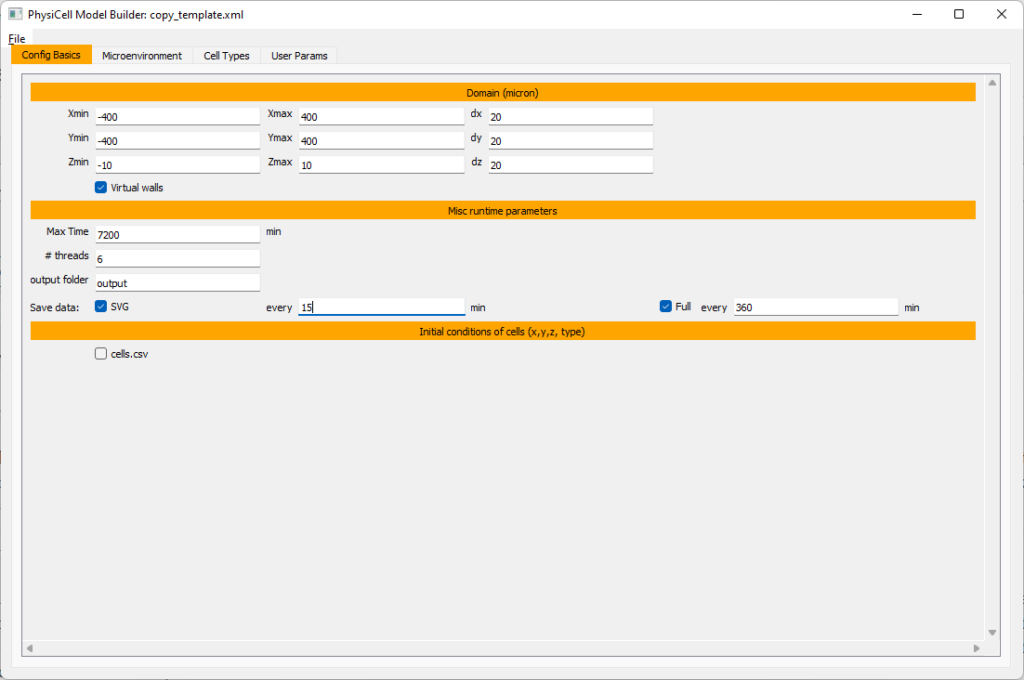#### Setting up diffusing substrates

Move to the microenvironment tab. Click on substrate and rename it to tumor signal . Set the decay rate to 0.1 and diffusion parameter to 10000. Uncheck the Dirichlet condition box so that it’s a zero-flux (Neumann) boundary condition.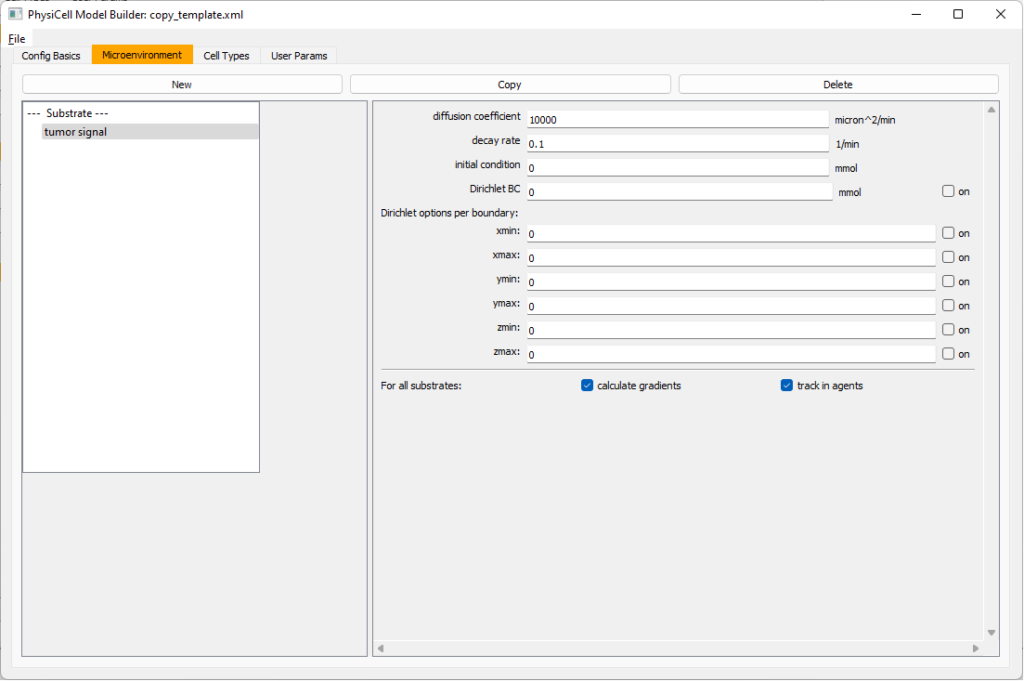Now, select tumor signal in the lefthand column, click copy, and rename the new substrate (substrate01) to pro-inflammatory signal with the same parameters.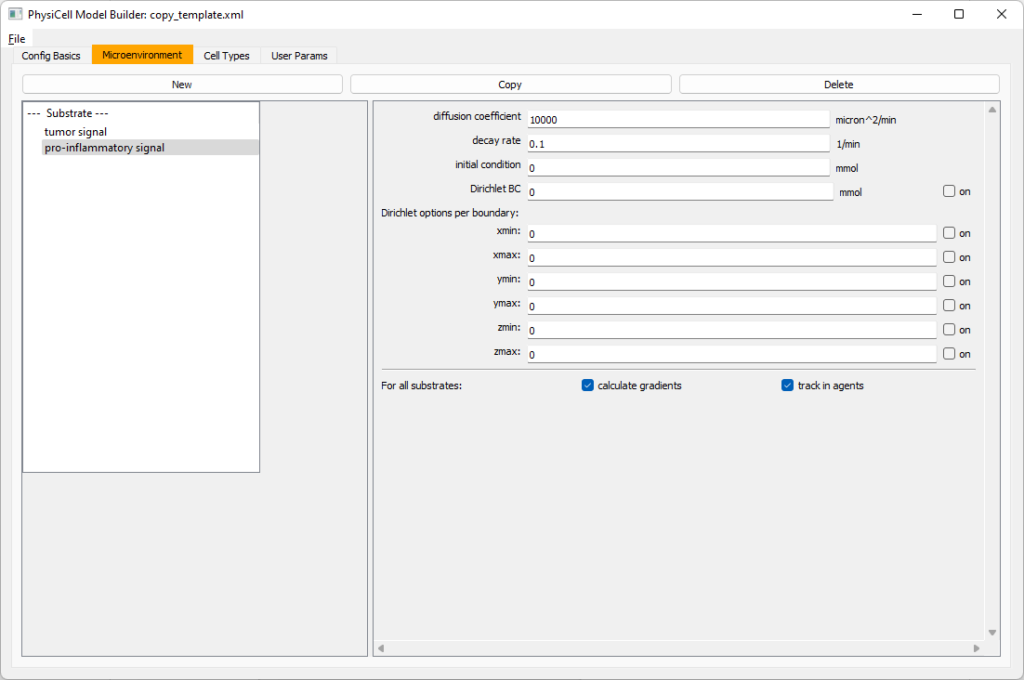Then, copy this substrate one more time, and rename it from name debris. Set its decay rate to 0.01, and its diffusion parameter to 1.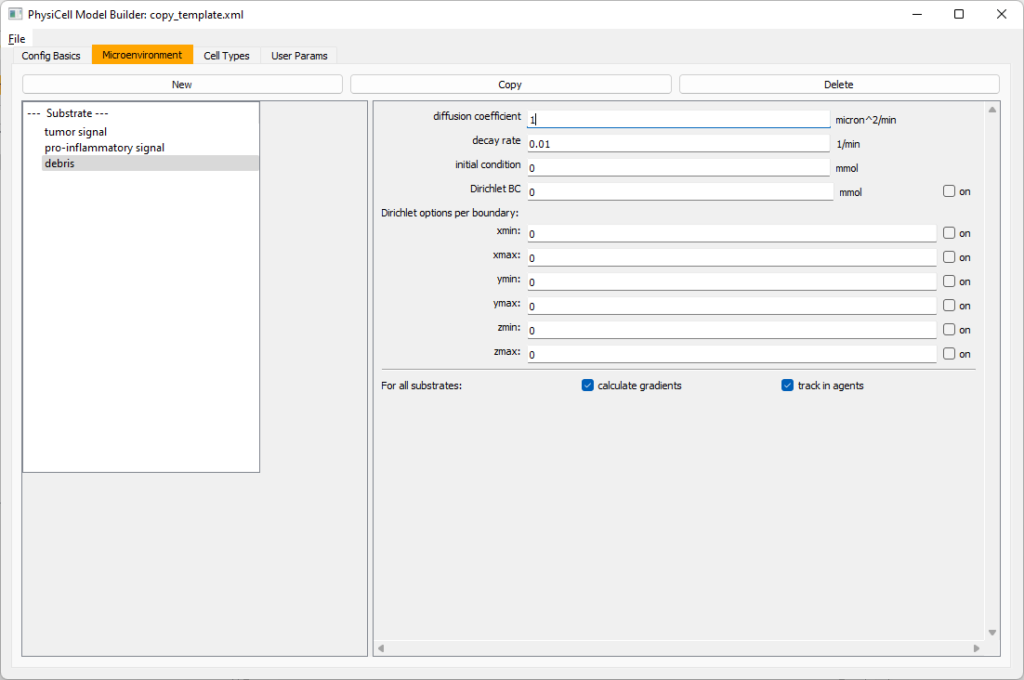Let’s save our work before continuing. Go to File, Save as, and save it as PhysiCell_settings.xml in your config file. (Go ahead and overwrite.)

If model builder crashes, you can reopen it, then go to File, Open, and select this file to continue editing.

#### Setting up the WT cancer cells

Move to the cell types tab. Click on the default cell type on the left side, and rename it to WT cancer cell. Leave most of the parameter values at their defaults. Move to the secretion sub-tab (in phenotype). From the drop-down menu, select tumor signal. Let’s set a high secretion rate of 10.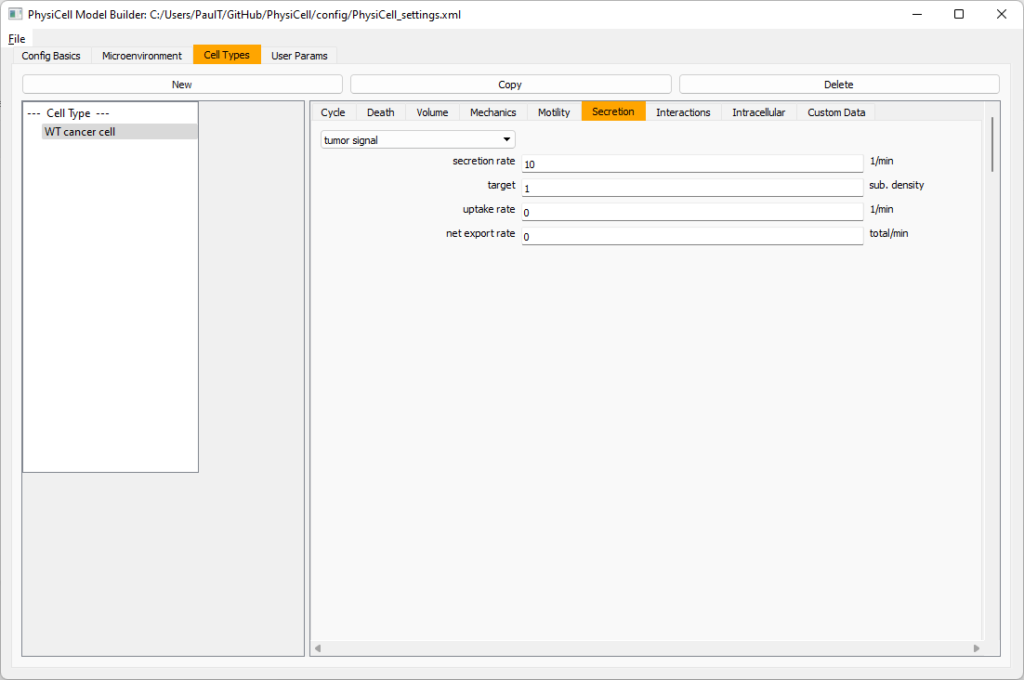We’ll need to set WT cells as able to mutate, but before that, the model builder needs to “know” about that cell type. So, let’s move on to the next cell type for now.

Setting up mutant tumor cells

Click the WT cancer cell in the left column, and click copy. Rename this cell type (for me, it’s cell_def03) to mutant cancer cell.  Click the secretion sub-tab, and set its secretion rate of tumor signal lower to 0.1.

#### Set the WT mutation rate

Now that both cell types are in the simulation, we can set up mutation. Let’s suppose the mean time to a mutation is 10000 minutes, or a rate of 0.0001 min$$^{-1}$$.

Click the WT cancer cell in the left column to select it. Go to the interactions tab. Go down to transformation rate, and choose mutant cancer cell from the drop-down menu. Set its rate to 0.0001.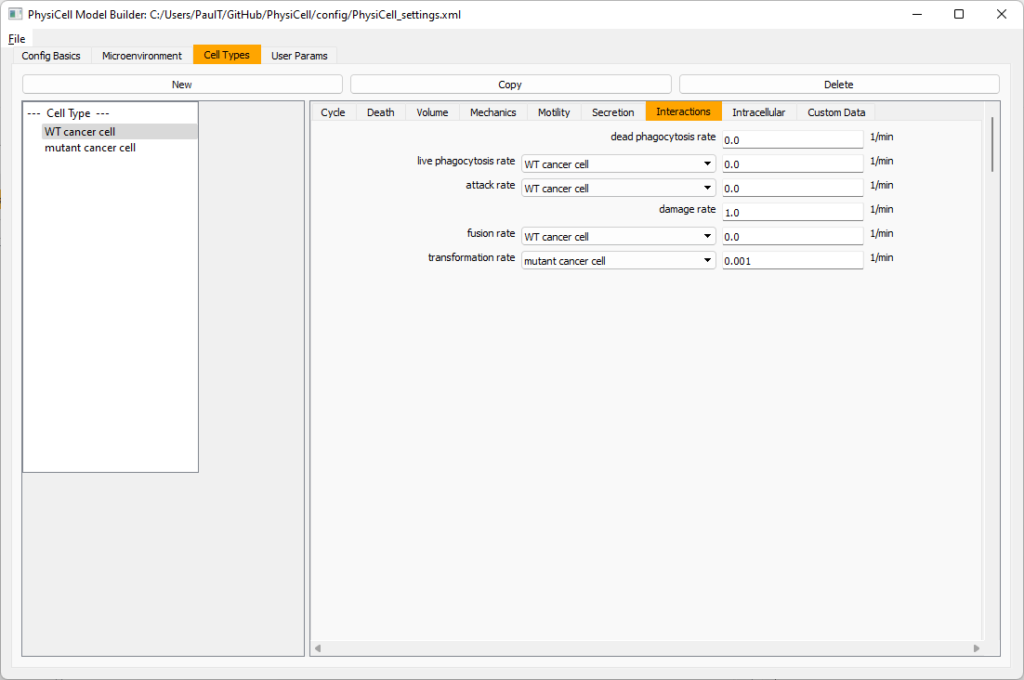#### Create macrophages

Now click on the WT cancer cell type in the left column, and click copy to create a new cell type. Click on the new type (for me cell_def04) and rename it to macrophage.

Click on cycle and change to a live cells and change from a duration representation to a transition rate representation. Keep its cycling rate at 0.

Go to death and change from duration to transition rate for both apoptosis and necrosis, and keep the death rates at 0.0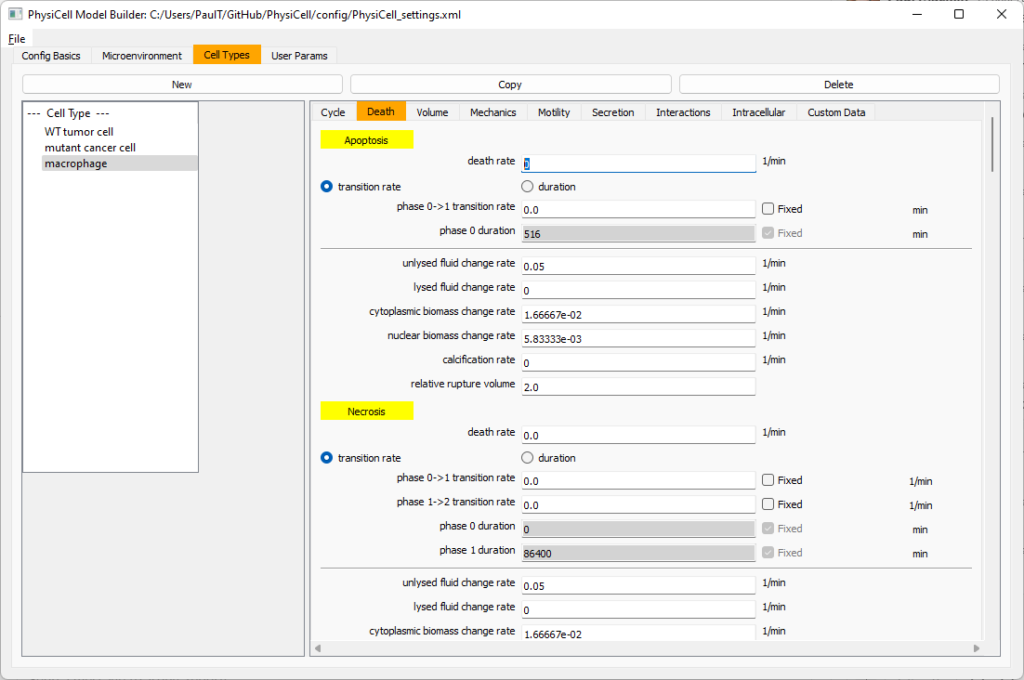Now, go to the motility tab. Set the speed to 1 $$\mu m/$$min, persistence time to 5 min, and migration bias to 0.5. Be sure to enable motility. Then, enable advanced motility. Choose tumor signal from the drop-down and set its sensitivity to 1.0. Then choose debris from the drop-down and set its sensitivity to 0.1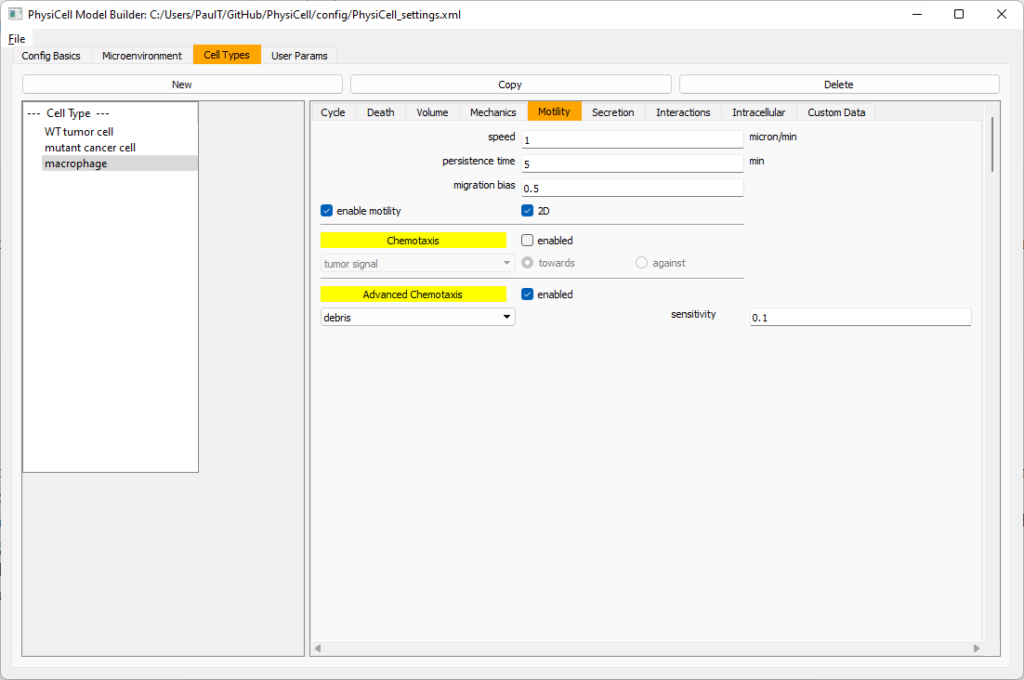Now, let’s make sure it secretes pro-inflammatory signal. Go to the secretion tab. Choose tumor signal from the drop-down, and make sure its secretion rate is 0. Then choose pro-inflammatory signal from the drop-down, set its secretion rate to 100, and its target value to 1.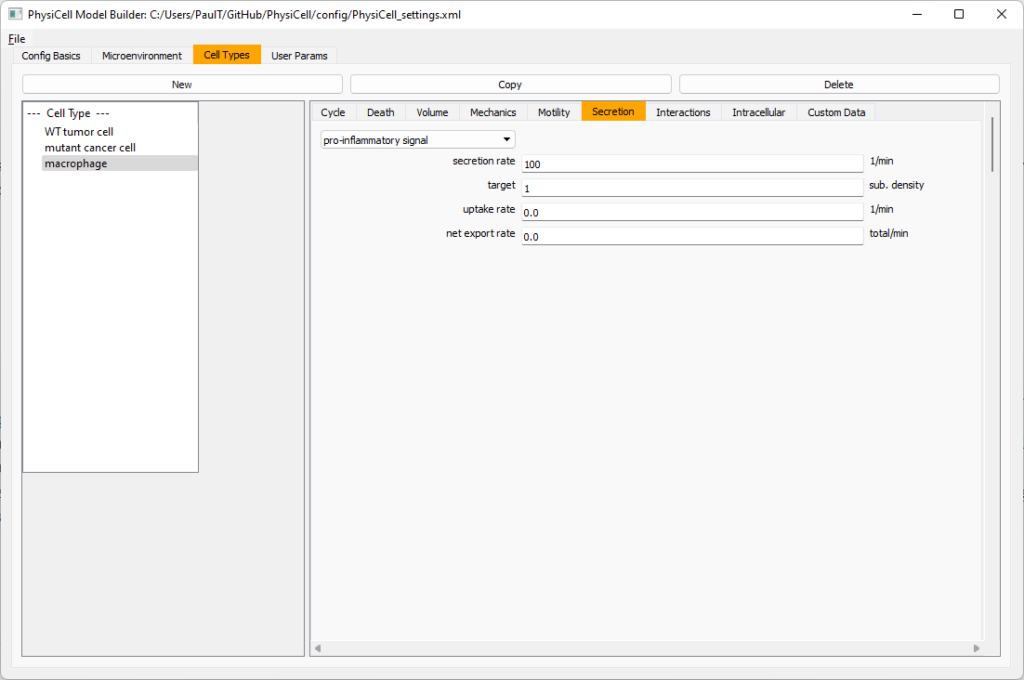Lastly, let’s set macrophages up to phagocytose dead cells. Go to the interactions tab. Set the dead phagocytosis rate to 0.05 (so that the mean contact time to wait to eat a dead cell is 1/0.05 = 20 minutes). This would be a good time to save your work.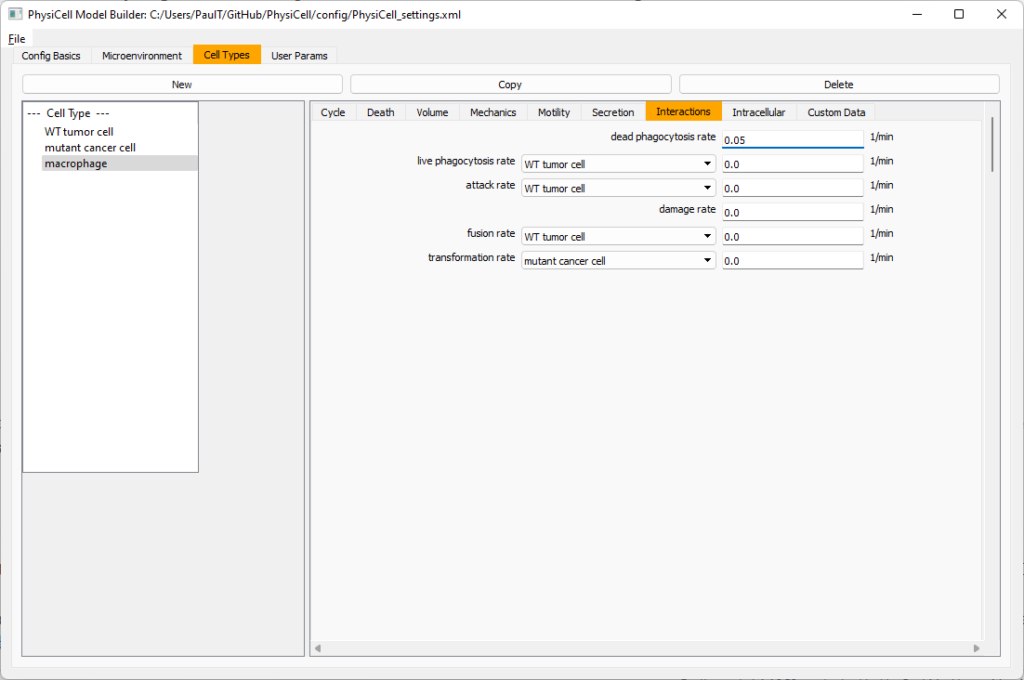Macrophages probably shouldn’t be adhesive. So go to the mechanics sub-tab, and set the cell-cell adhesion strength to 0.0.

#### Create CD8+ T cells

Select macrophage on the left column, and choose copy. Rename the new cell type to CD8+ T cell.  Go to the secretion sub-tab and make sure to set the secretion rate of pro-inflammatory signal to 0.0.

Now, let’s use simpler chemotaxis for these towards pro-inflammatory signal. Go to the motility tab. Uncheck advanced chemotaxis and check chemotaxis. Choose pro-inflammatory signal from the drop-down, with the towards option.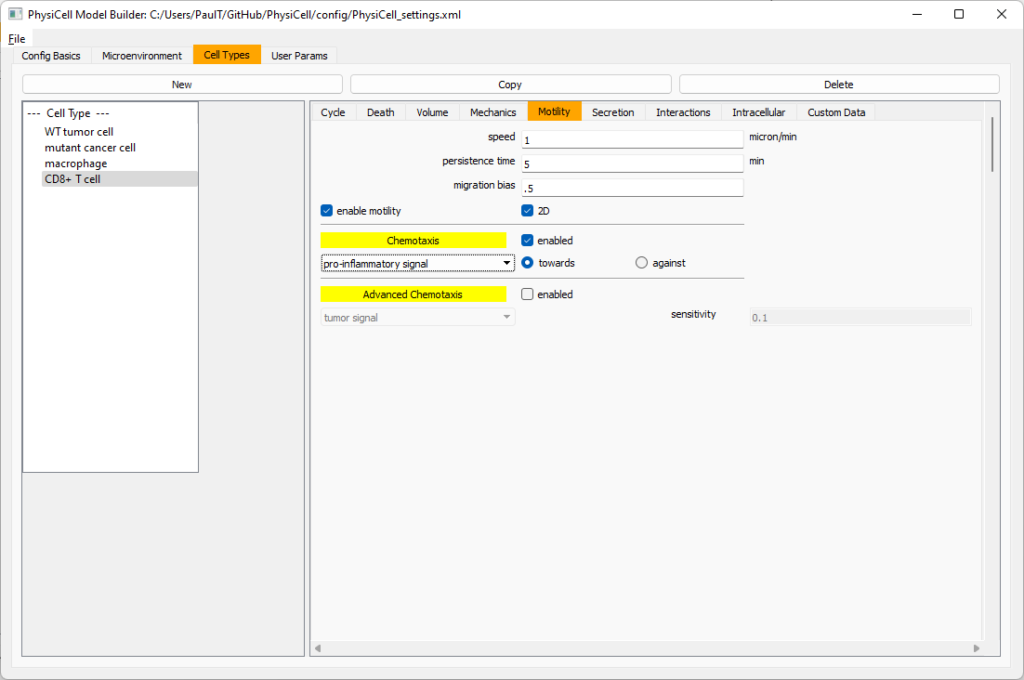Lastly, let’s set these cells to attack WT and tumor cells. Go to the interactions tab. Set the dead phagocytosis rate to 0.

Now, let’s work on attack. Set the damage rate to 1.0. Near attack rate, choose WT tumor cell from the drop-down, and set its attack rate to 10 (so it attacks during any 0.1 minute interval). Then choose mutant tumor cell from the drop-down, and set its attack rate to 1 (as a model of immune evasion, where they are less likely to attack a non-WT tumor cell).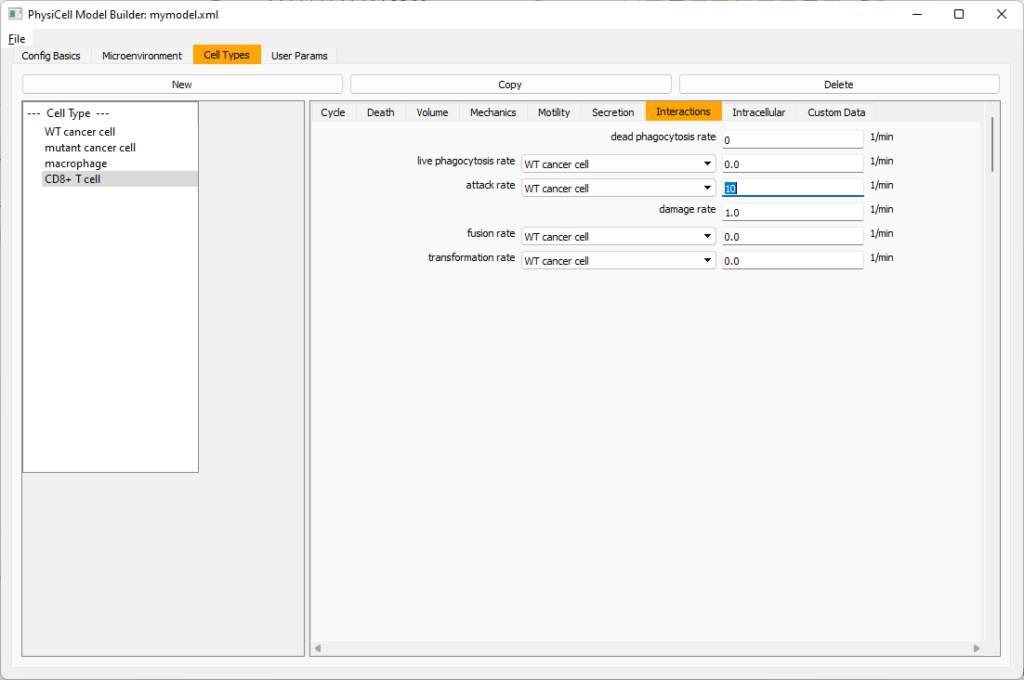#### Set the initial cell conditions

Go to the user params tab. On number of cells, choose 50. It will randomly place 50 of each cell type at the start of the simulation.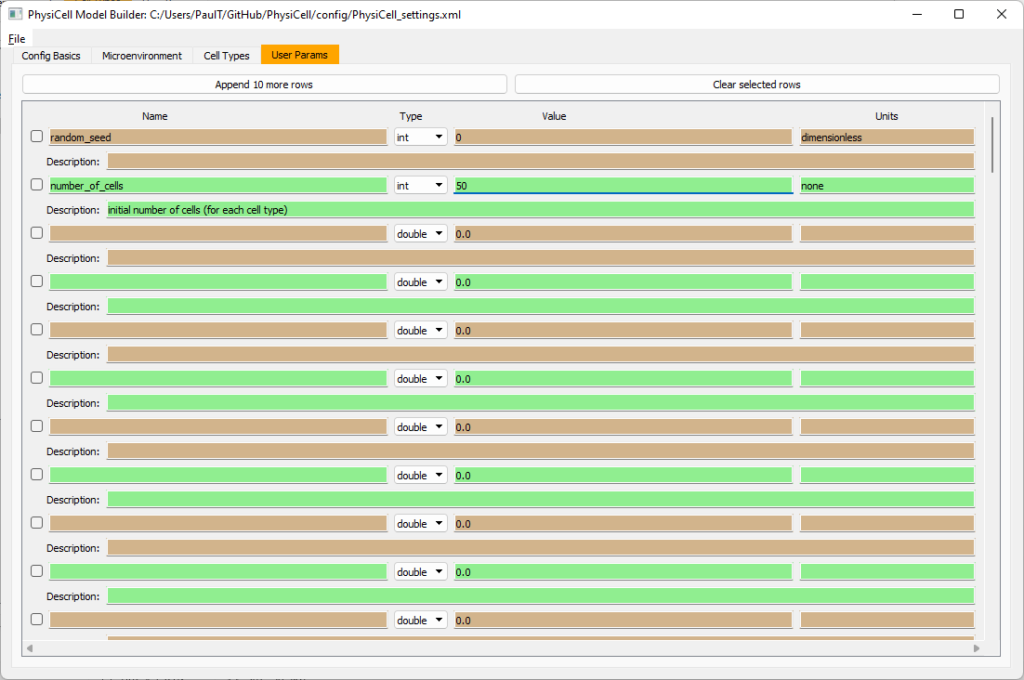That’s all! Go to File and save (or save as), and overwrite config/PhysiCell_settings.xml. Then close the model builder.

#### Testing the model

Go into the PhysiCell directory. Since you already compiled, just run your model (which will use PhysiCell_settings.xml that you just saved to the config directory). For Linux or MacOS (or similar Unix-like systems):

./project


Windows users would type project without the ./ at the front.

Take a look at your SVG outputs in the output directory. You might consider making an animated gif (make gif) or a movie:

make jpeg && make movie


Here’s what we have.

Promising! But we still need to write a little C++ so that damage kills tumor cells, and so that dead tumor cells release debris. (And we could stand to “anchor” tumor cells to ECM so they don’t get pushed around by the immune cells, but that’s a post for another day!)

#### Customizing the WT and mutant tumor cells with phenotype functions

First, open custom.h in the custom_modules directory, and declare a function for tumor phenotype:

void tumor_phenotype_function( Cell* pCell, Phenotype& phenotype, double dt );


Now, let’s open custom.cpp to write this function at the bottom. Here’s what we’ll do:

• If dead, set rate of debris release to 1.0 and return.
• Otherwise, we’ll set damage-based apoptosis.
• Get the base apoptosis rate
• Get the current damage
• Use a Hill response function with a half-max of 36, max apoptosis rate of 0.05 min$$^{-1}$$, and a hill power of 2.0

Here’s the code:

void tumor_phenotype_function( Cell* pCell, Phenotype& phenotype, double dt )
{
// find my cell definition
static Cell_Definition* pCD = find_cell_definition( pCell->type_name );

// find the index of debris in the environment
static int nDebris = microenvironment.find_density_index( "debris" );
static int nTS = microenvironment.find_density_index( "tumor signal" );

// if dead: release debris. stop releasing tumor signal
{
phenotype.secretion.secretion_rates[nDebris] = 1;
phenotype.secretion.saturation_densities[nDebris] = 1;

phenotype.secretion.secretion_rates[nTS] = 0;

// last time I'll execute this function (special optional trick)
pCell->functions.update_phenotype = NULL;
return;
}

// damage increases death

// find death model
static int nApoptosis =
phenotype.death.find_death_model_index( PhysiCell_constants::apoptosis_death_model );

double signal = pCell->state.damage; // current damage
double base_val = pCD->phenotype.death.rates[nApoptosis]; // base death rate (from cell def)
double max_val = 0.05; // max death rate (at large damage)

static double damage_halfmax = 36.0;
double hill_power = 2.0;

// evaluate Hill response function
double hill = Hill_response_function( signal , damage_halfmax , hill_power );
// set "dose-dependent" death rate
phenotype.death.rates[nApoptosis] = base_val + (max_val-base_val)*hill;

return;
}


Now, we need to make sure we apply these functions to tumor cell. Go to create_cell_types and look just before we display the cell definitions. We need to:

• Search for the WT tumor cell definition
• Set the update_phenotype function for that type to the tumor_phenotype_function we just wrote
• Repeat for the mutant tumor cell type.

Here’s the code:

...
/*
Put any modifications to individual cell definitions here.

This is a good place to set custom functions.
*/

cell_defaults.functions.update_phenotype = phenotype_function;
cell_defaults.functions.custom_cell_rule = custom_function;
cell_defaults.functions.contact_function = contact_function;

Cell_Definition* pCD = find_cell_definition( "WT cancer cell");
pCD->functions.update_phenotype = tumor_phenotype_function;

pCD = find_cell_definition( "mutant cancer cell");
pCD->functions.update_phenotype = tumor_phenotype_function;

/*
This builds the map of cell definitions and summarizes the setup.
*/

display_cell_definitions( std::cout );
return;
}


That’s it! Let’s recompile and run!

make && ./project


And here’s the final movie.

Much better! Now the

### Coming up next!

We will use the signal and behavior dictionaries to help us easily write C++ functions to modulate the tumor and immune cell behaviors.

Once ready, this will be posted at http://www.mathcancer.org/blog/introducing-cell-signal-and-behavior-dictionaries/.

Tags :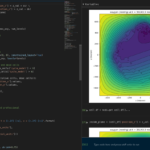The newest tool for PhysiCell provides an easy way to load your PhysiCell output data into python for analysis. This builds upon previous work on loading data into MATLAB. A post on that tool can be found at:

PhysiCell stores output data as a MultiCell Digital Snapshot (MultiCellDS) that consists of several files for each time step and is probably stored in your ./output directory. pyMCDS is a python object that is initialized with the .xml file

### What you’ll need

• python-loader, available on GitHub at
• Python 3.x, recommended distribution available at
• A number of Python packages, included in anaconda or available through pip
• NumPy
• pandas
• scipy
• Some PhysiCell data, probably in your ./output directory

### Anatomy of a MultiCell Digital Snapshot

Each time PhysiCell’s internal time tracker passes a time step where data is to be saved, it generates a number of files of various types. Each of these files will have a number at the end that indicates where it belongs in the sequence of outputs. All of the files from the first round of output will end in 00000000.* and the second round will be 00000001.* and so on. Let’s say we’re interested in a set of output from partway through the run, the 88th set of output files. The files we care about most from this set consists of:

• output00000087.xml: This file is the main organizer of the data. It contains an overview of the data stored in the MultiCellDS as well as some actual data including:
• Metadata about the time and runtime for the current time step
• Coordinates for the computational domain
• Parameters for diffusing substrates in the microenvironment
• Column labels for the cell data
• File names for the files that contain microenvironment and cell data at this time step
• output00000087_microenvironment0.mat: This is a MATLAB matrix file that contains all of the data about the microenvironment at this time step
• output00000087_cells_physicell.mat: This is a MATLAB matrix file that contains all of the tracked information about the individual cells in the model. It tells us things like the cells’ position, volume, secretion, cell cycle status, and user-defined cell parameters.

### Setup

#### Using pyMCDS

From the appropriate file in your PhysiCell directory, wherever pyMCDS.py lives, you can use the data loader in your own scripts or in an interactive session. To start you have to import the pyMCDS class

from pyMCDS import pyMCDS


Data is loaded into python from the MultiCellDS by initializing the pyMCDS object. The initialization function for pyMCDS takes one required and one optional argument.

__init__(xml_file, [output_path = '.'])
'''
xml_file : string
String containing the name of the output xml file
output_path :
String containing the path (relative or absolute) to the directory
where PhysiCell output files are stored
'''


We are interested in reading output00000087.xml that lives in ~/path/to/PhysiCell/output (don’t worry Windows paths work too). We would initialize our pyMCDS object using those names and the actual data would be stored in a member dictionary called data.

mcds = pyMCDS('output00000087.xml', '~/path/to/PhysiCell/output')
# Now our data lives in:
mcds.data


We’ve tried to keep everything organized inside of this dictionary but let’s take a look at what we actually have in here. Of course in real output, there will probably not be a chemical named my_chemical, this is simply there to illustrate how multiple chemicals are handled.The data member dictionary is a dictionary of dictionaries whose child dictionaries can be accessed through normal python dictionary syntax.

mcds.data['metadata']
mcds.data['continuum_variables']['my_chemical']


Each of these subdictionaries contains data, we will take a look at exactly what that data is and how it can be accessed in the following sections.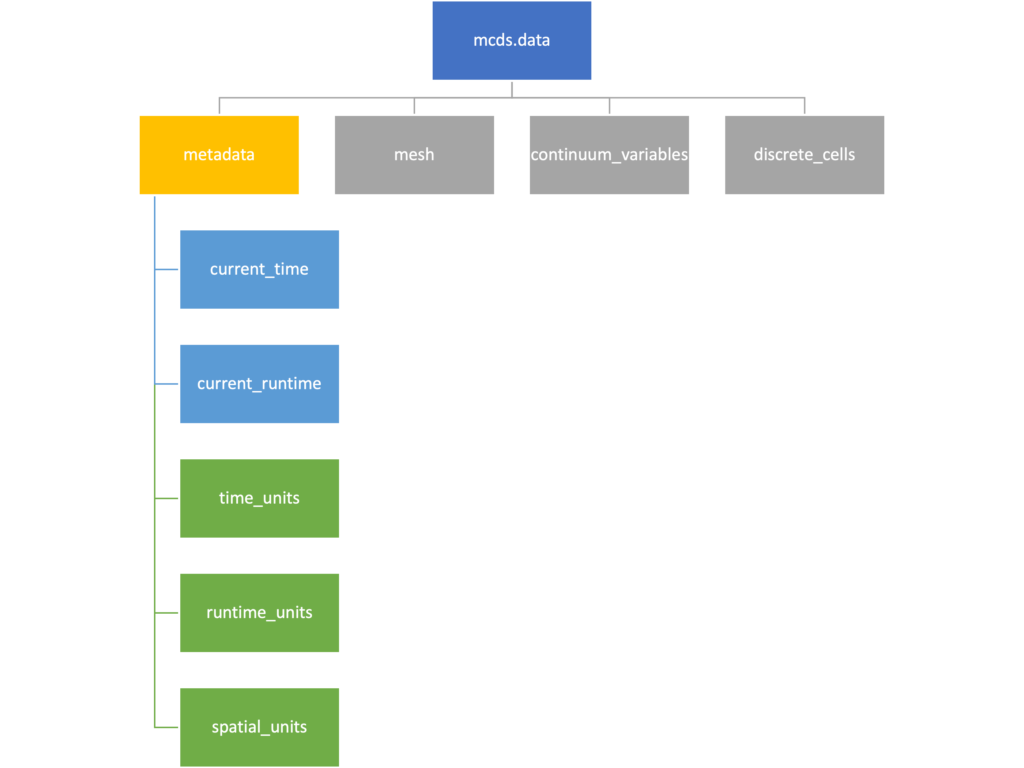The metadata dictionary contains information about the time of the simulation as well as units for both times and space. Here and in later sections blue boxes indicate scalars and green boxes indicate strings. We can access each of these things using normal dictionary syntax. We’ve also got access to a helper function get_time() for the common operation of retrieving the simulation time.

>>> mcds.data['metadata']['time_units']
'min'
>>> mcds.get_time()
5220.0


#### Mesh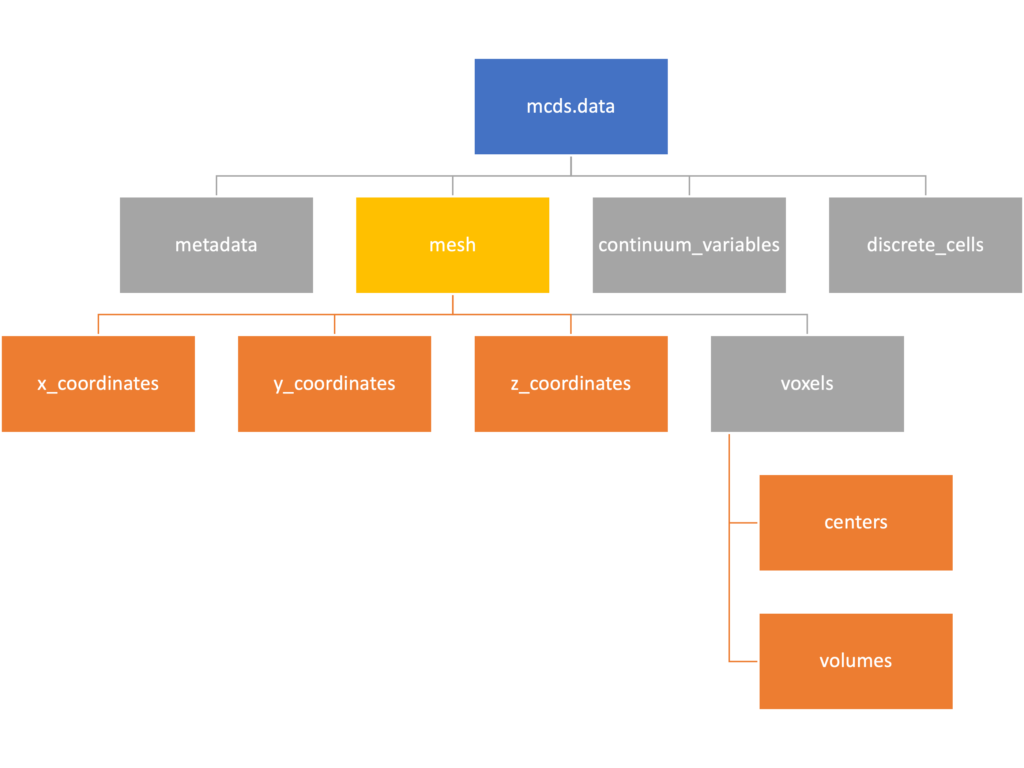The mesh dictionary has a lot more going on than the metadata dictionary. It contains three numpy arrays, indicated by orange boxes, as well as another dictionary. The three arrays contain $$x$$, $$y$$ and $$z$$ coordinates for the centers of the voxels that constiture the computational domain in a meshgrid format. This means that each of those arrays is tensors of rank three. Together they identify the coordinates of each possible point in the space.

In contrast, the arrays in the voxel dictionary are stored linearly. If we know that we care about voxel number 42, we want to use the stuff in the voxels dictionary. If we want to make a contour plot, we want to use the x_coordinates, y_coordinates, and z_coordinates arrays.

# We can extract one of the meshgrid arrays as a numpy array
>>> y_coords = mcds.data['mesh']['y_coordinates']
>>> y_coords.shape
(75, 75, 75)
>>> y_coords[0, 0, :4]
array([-740., -740., -740., -740.])

# We can also extract the array of voxel centers
>>> centers = mcds.data['mesh']['voxels']['centers']
>>> centers.shape
(3, 421875)
>>> centers[:, :4]
array([[-740., -720., -700., -680.],
[-740., -740., -740., -740.],
[-740., -740., -740., -740.]])

# We have a handy function to quickly extract the components of the full meshgrid
>>> xx, yy, zz = mcds.get_mesh()
>>> yy.shape
(75, 75, 75)
>>> yy[0, 0, :4]
array([-740., -740., -740., -740.])

# We can also use this to return the meshgrid describing an x, y plane
>>> xx, yy = mcds.get_2D_mesh()
>>> yy.shape
(75, 75)


#### Continuum variables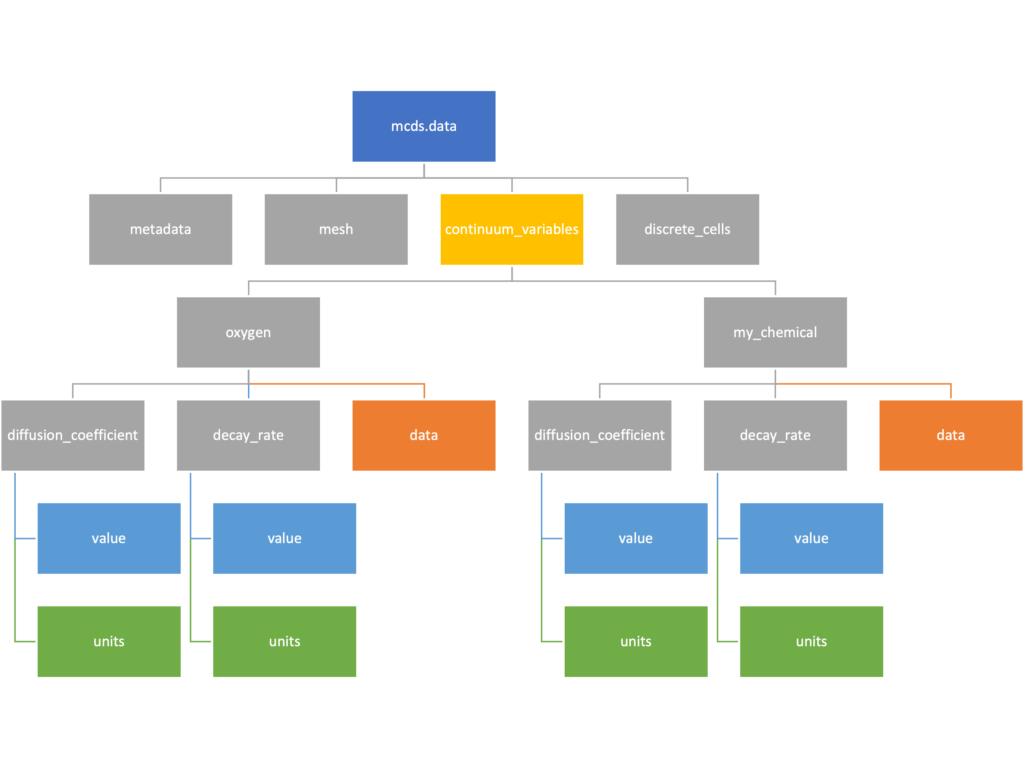The continuum_variables dictionary is the most complicated of the four. It contains subdictionaries that we access using the names of each of the chemicals in the microenvironment. In our toy example above, these are oxygen and my_chemical. If our model tracked diffusing oxygen, VEGF, and glucose, then the continuum_variables dictionary would contain a subdirectory for each of them.

For a particular chemical species in the microenvironment we have two more dictionaries called decay_rate and diffusion_coefficient, and a numpy array called data. The diffusion and decay dictionaries each complete the value stored as a scalar and the unit stored as a string. The numpy array contains the concentrations of the chemical in each voxel at this time and is the same shape as the meshgrids of the computational domain stored in the .data[‘mesh’] arrays.

# we need to know the names of the substrates to work with
# this data. We have a function to help us find them.
>>> mcds.get_substrate_names()
['oxygen', 'my_chemical']

# The diffusable chemical dictionaries are messy
# if we need to do a lot with them it might be easier
# to put them into their own instance
>>> oxy_dict = mcds.data['continuum_variables']['oxygen']
>>> oxy_dict['decay_rate']
{'value': 0.1, 'units': '1/min'}

# What we care about most is probably the numpy
# array of concentrations
>>> oxy_conc = oxy_dict['data']
>>> oxy_conc.shape
(75, 75, 75)

# Alternatively, we can get the same array with a function
>>> oxy_conc2 = mcds.get_concentrations('oxygen')
>>> oxy_conc2.shape
(75, 75, 75)

# We can also get the concentrations on a plane using the
# same function and supplying a z value to "slice through"
# note that right now the z_value must be an exact match
# for a plane of voxel centers, in the future we may add
# interpolation.
>>> oxy_plane = mcds.get_concentrations('oxygen', z_value=100.0)
>>> oxy_plane.shape
(75, 75)

# we can also find the concentration in a single voxel using the
# position of a point within that voxel. This will give us an
# array of all concentrations at that point.
>>> mcds.get_concentrations_at(x=0., y=550., z=0.)
array([17.94514446,  0.99113448])


#### Discrete Cells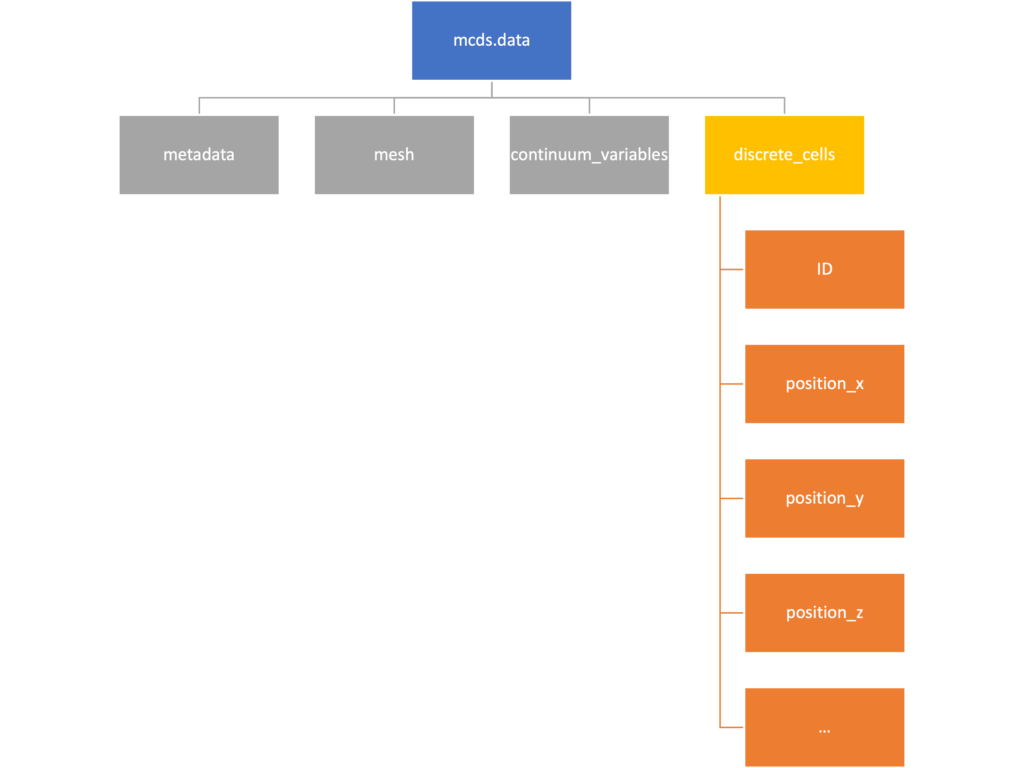The discrete cells dictionary is relatively straightforward. It contains a number of numpy arrays that contain information regarding individual cells.  These are all 1-dimensional arrays and each corresponds to one of the variables specified in the output*.xml file. With the default settings, these are:

• ID: unique integer that will identify the cell throughout its lifetime in the simulation
• position(_x, _y, _z): floating point positions for the cell in $$x$$, $$y$$, and $$z$$ directions
• total_volume: total volume of the cell
• cell_type: integer label for the cell as used in PhysiCell
• cycle_model: integer label for the cell cycle model as used in PhysiCell
• current_phase: integer specification for which phase of the cycle model the cell is currently in
• elapsed_time_in_phase: time that cell has been in current phase of cell cycle model
• nuclear_volume: volume of cell nucleus
• cytoplasmic_volume: volume of cell cytoplasm
• fluid_fraction: proportion of the volume due to fliud
• calcified_fraction: proportion of volume consisting of calcified material
• orientation(_x, _y, _z): direction in which cell is pointing
• polarity:
• migration_speed: current speed of cell
• motility_vector(_x, _y, _z): current direction of movement of cell
• migration_bias: coefficient for stochastic movement (higher is “more deterministic”)
• motility_bias_direction(_x, _y, _z): direction of movement bias
• persistence_time: time in-between direction changes for cell
• motility_reserved:
# Extracting single variables is just like before
>>> cell_ids = mcds.data['discrete_cells']['ID']
>>> cell_ids.shape
(18595,)
>>> cell_ids[:4]
array([0., 1., 2., 3.])

# If we're clever we can extract 2D arrays
>>> cell_vec = np.zeros((cell_ids.shape, 3))
>>> vec_list = ['position_x', 'position_y', 'position_z']
>>> for i, lab in enumerate(vec_list):
...     cell_vec[:, i] = mcds.data['discrete_cells'][lab]
...
array([[ -69.72657128,  -39.02046405, -233.63178904],
[ -69.84507464,  -22.71693265, -233.59277388],
[ -69.84891462,   -6.04070516, -233.61816711],
[ -69.845265  ,   10.80035554, -233.61667313]])

# We can get the list of all of the variables stored in this dictionary
>>> mcds.get_cell_variables()
['ID',
'position_x',
'position_y',
'position_z',
'total_volume',
'cell_type',
'cycle_model',
'current_phase',
'elapsed_time_in_phase',
'nuclear_volume',
'cytoplasmic_volume',
'fluid_fraction',
'calcified_fraction',
'orientation_x',
'orientation_y',
'orientation_z',
'polarity',
'migration_speed',
'motility_vector_x',
'motility_vector_y',
'motility_vector_z',
'migration_bias',
'motility_bias_direction_x',
'motility_bias_direction_y',
'motility_bias_direction_z',
'persistence_time',
'motility_reserved',
'oncoprotein',
'elastic_coefficient',
'kill_rate',
'attachment_rate']
# We can also get all of the cell data as a pandas DataFrame
>>> cell_df = mcds.get_cell_df()
ID     position_x   position_y    position_z total_volume cell_type cycle_model ...
0.0   - 69.726571  - 39.020464  - 233.631789       2494.0       0.0         5.0 ...
1.0   - 69.845075  - 22.716933  - 233.592774       2494.0       0.0         5.0 ...
2.0   - 69.848915  - 6.040705   - 233.618167       2494.0       0.0         5.0 ...
3.0   - 69.845265    10.800356  - 233.616673       2494.0       0.0         5.0 ...
4.0   - 69.828161    27.324530  - 233.631579       2494.0       0.0         5.0 ...

# if we want to we can also get just the subset of cells that
# are in a specific voxel
>>> vox_df = mcds.get_cell_df_at(x=0.0, y=550.0, z=0.0)
>>> vox_df.iloc[:, :5]
ID  position_x  position_y  position_z  total_volume
26718  228761.0    6.623617  536.709341   -1.282934   2454.814507
52736  270274.0   -7.990034  538.184921    9.648955   1523.386488


### Examples

These examples will not be made using our toy dataset described above but will instead be made using a single timepoint dataset that can be found at:

#### Substrate contour plot

One of the big advantages of working with PhysiCell data in python is that we have access to its plotting tools. For the sake of example let’s plot the partial pressure of oxygen throughout the computational domain along the $$z = 0$$ plane. Once we’ve loaded our data by initializing a pyMCDS object, we can work entirely within python to produce the plot.

from pyMCDS import pyMCDS
import numpy as np
import matplotlib.pyplot as plt

mcds = pyMCDS('output00003696.xml', '../output')

# Set our z plane and get our substrate values along it
z_val = 0.00
plane_oxy = mcds.get_concentrations('oxygen', z_slice=z_val)

# Get the 2D mesh for contour plotting
xx, yy = mcds.get_mesh()

# We want to be able to control the number of contour levels so we
# need to do a little set up
num_levels = 21
min_conc = plane_oxy.min()
max_conc = plane_oxy.max()
my_levels = np.linspace(min_conc, max_conc, num_levels)

# set up the figure area and add data layers
fig, ax = plt.subplot()
cs = ax.contourf(xx, yy, plane_oxy, levels=my_levels)
ax.contour(xx, yy, plane_oxy, color='black', levels = my_levels,
linewidths=0.5)

# Now we need to add our color bar
cbar1 = fig.colorbar(cs, shrink=0.75)
cbar1.set_label('mmHg')

# Let's put the time in to make these look nice
ax.set_aspect('equal')
ax.set_xlabel('x (micron)')
ax.set_ylabel('y (micron)')
ax.set_title('oxygen (mmHg) at t = {:.1f} {:s}, z = {:.2f} {:s}'.format(
mcds.get_time(),
z_val,

plt.show()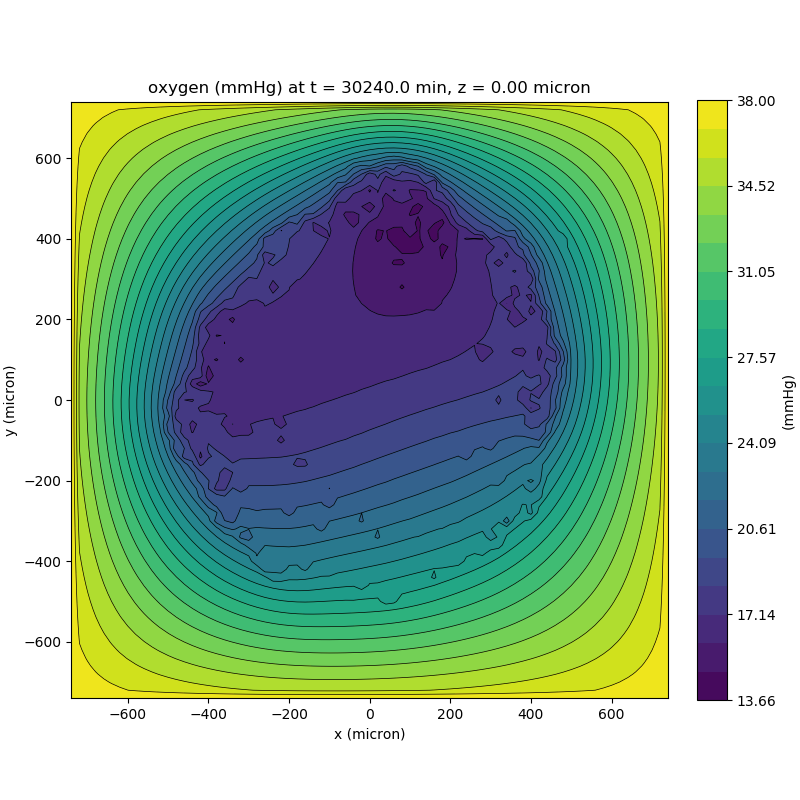We can also use pandas to do fairly complex selections of cells to add to our plots. Below we use pandas and the previous plot to add a cells layer.

from pyMCDS import pyMCDS
import numpy as np
import matplotlib.pyplot as plt

mcds = pyMCDS('output00003696.xml', '../output')

# Set our z plane and get our substrate values along it
z_val = 0.00
plane_oxy = mcds.get_concentrations('oxygen', z_slice=z_val)

# Get the 2D mesh for contour plotting
xx, yy = mcds.get_mesh()

# We want to be able to control the number of contour levels so we
# need to do a little set up
num_levels = 21
min_conc = plane_oxy.min()
max_conc = plane_oxy.max()
my_levels = np.linspace(min_conc, max_conc, num_levels)

# get our cells data and figure out which cells are in the plane
cell_df = mcds.get_cell_df()
ds = mcds.get_mesh_spacing()
inside_plane = (cell_df['position_z'] < z_val + ds) \ & (cell_df['position_z'] > z_val - ds)
plane_cells = cell_df[inside_plane]

# We're going to plot two types of cells and we want it to look nice
colors = ['black', 'grey']
sizes = [20, 8]

# set up the figure area and add microenvironment layer
fig, ax = plt.subplot()
cs = ax.contourf(xx, yy, plane_oxy, levels=my_levels)

# get our cells of interest
# alive_cells = plane_cells[plane_cells['cycle_model'] < 6]
# dead_cells = plane_cells[plane_cells['cycle_model'] > 6]
# -- for newer versions of PhysiCell
alive_cells = plane_cells[plane_cells['cycle_model'] < 100]

# plot the cell layer
for i, plot_cells in enumerate((alive_cells, dead_cells)):
ax.scatter(plot_cells['position_x'].values,
plot_cells['position_y'].values,
facecolor='none',
edgecolors=colors[i],
alpha=0.6,
s=sizes[i],
label=labels[i])

# Now we need to add our color bar
cbar1 = fig.colorbar(cs, shrink=0.75)
cbar1.set_label('mmHg')

# Let's put the time in to make these look nice
ax.set_aspect('equal')
ax.set_xlabel('x (micron)')
ax.set_ylabel('y (micron)')
ax.set_title('oxygen (mmHg) at t = {:.1f} {:s}, z = {:.2f} {:s}'.format(
mcds.get_time(),
z_val,
ax.legend(loc='upper right')

plt.show()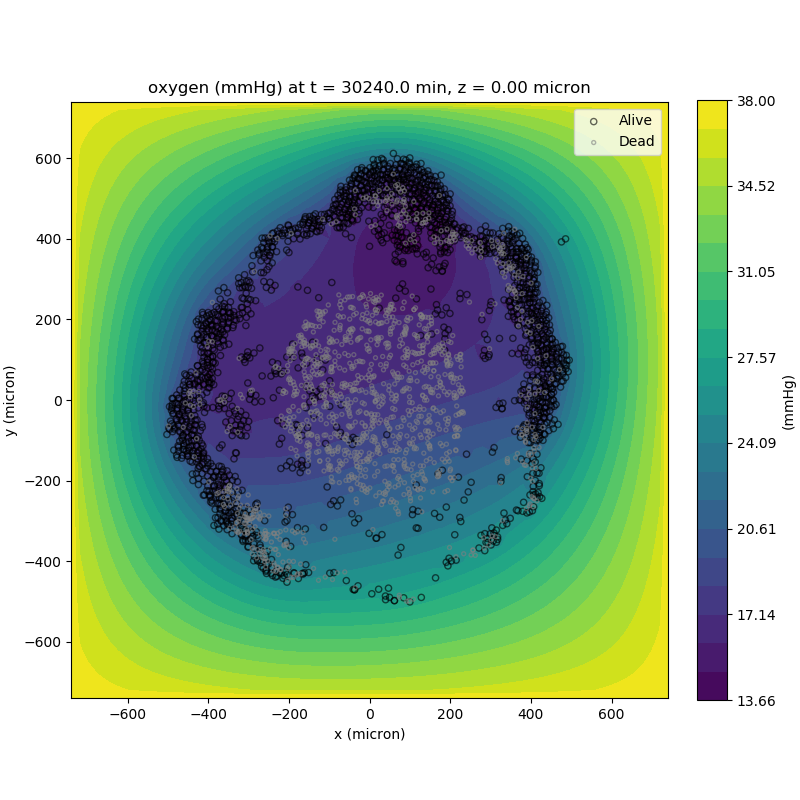#### Future Direction

The first extension of this project will be timeseries functionality. This will provide similar data loading functionality but for a time series of MultiCell Digital Snapshots instead of simply one point in time.

## PhysiCell Tools : PhysiCell-povwriter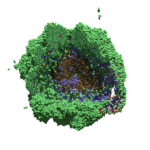As PhysiCell matures, we are starting to turn our attention to better training materials and an ecosystem of open source PhysiCell tools. PhysiCell-povwriter is is designed to help transform your 3-D simulation results into 3-D visualizations like this one:

PhysiCell-povwriter transforms simulation snapshots into 3-D scenes that can be rendered into still images using POV-ray: an open source software package that uses raytracing to mimic the path of light from a source of illumination to a single viewpoint (a camera or an eye). The result is a beautifully rendered scene (at any resolution you choose) with very nice shading and lighting.

If you repeat this on many simulation snapshots, you can create an animation of your work.

### What you’ll need

This workflow is entirely based on open source software:

• 3-D simulation data (likely stored in ./output from your project)
• PhysiCell-povwriter, available on GitHub at
• POV-ray, available at
• ImageMagick (optional, for image file conversions)
• mencoder (optional, for making compressed movies)

### Setup

#### Building PhysiCell-povwriter

After you clone PhysiCell-povwriter or download its source from a release, you’ll need to compile it. In the project’s root directory, compile the project by:

make


(If you need to set up a C++ PhysiCell development environment, click here for OSX or here for Windows.)

Next, copy povwriter (povwriter.exe in Windows) to either the root directory of your PhysiCell project, or somewhere in your path. Copy ./config/povwriter-settings.xml to the ./config directory of your PhysiCell project.

#### Editing resolutions in POV-ray

PhysiCell-povwriter is intended for creating “square” images, but POV-ray does not have any pre-created square rendering resolutions out-of-the-box. However, this is straightforward to fix.

1. Open POV-Ray
2. Go to the “tools” menu and select “edit resolution INI file”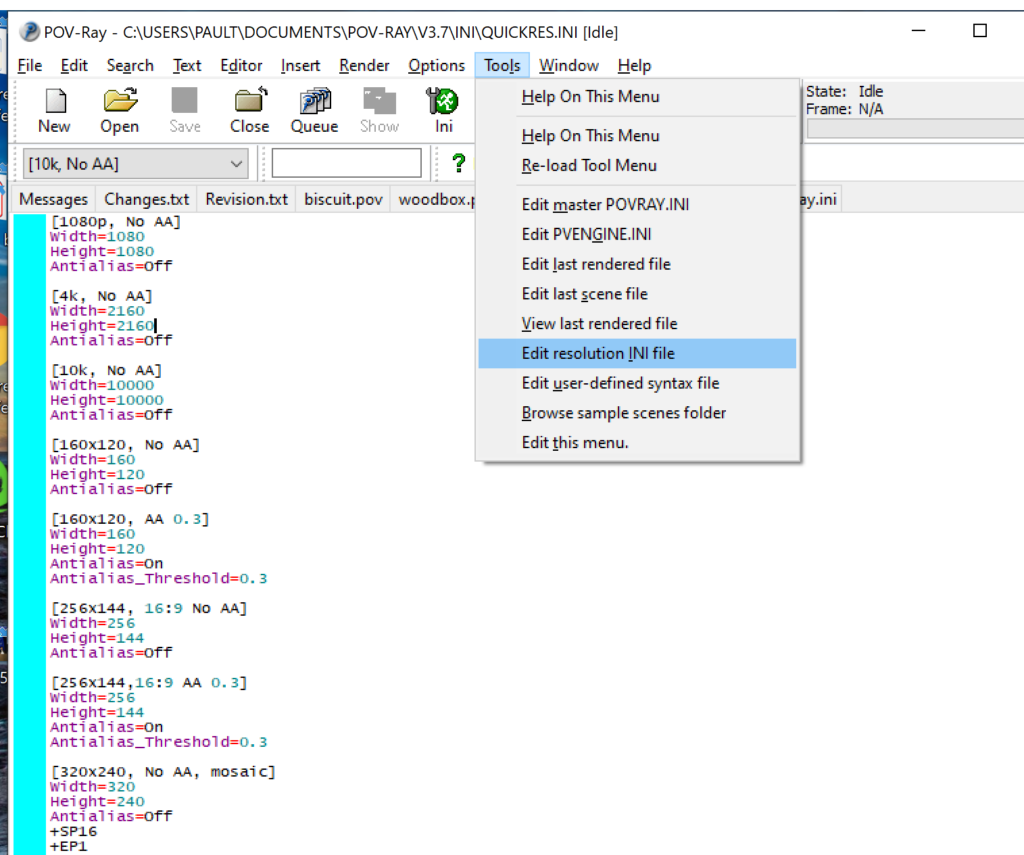3. At the top of the INI file (which opens for editing in POV-ray), make a new profile:
[1080x1080, AA]
Width=480
Height=480
Antialias=On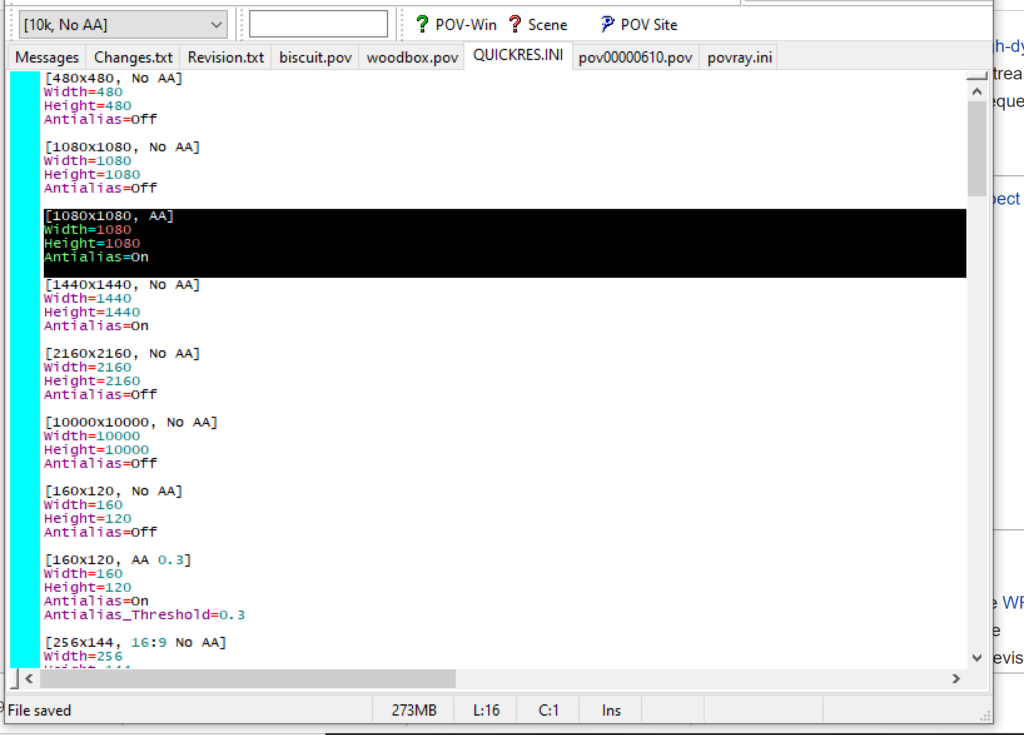4. Make similar profiles (with unique names) to suit your preferences. I suggest one at 480×480 (as a fast preview), another at 2160×2160, and another at 5000×5000 (because they will be absurdly high resolution). For example:
[2160x2160 no AA]
Width=2160
Height=2160
Antialias=Off


You can optionally make more profiles with antialiasing on (which provides some smoothing for areas of high detail), but you’re probably better off just rendering without antialiasing at higher resolutions and the scaling the image down as needed. Also, rendering without antialiasing will be faster.

5. Once done making profiles, save and exit POV-Ray.
6. The next time you open POV-Ray, your new resolution profiles will be available in the lefthand dropdown box.

### Configuring PhysiCell-povwriter

Once you have copied povwriter-settings.xml to your project’s config file, open it in a text editor. Below, we’ll show the different settings.

#### Camera settings

<camera>
<distance_from_origin units="micron">1500</distance_from_origin>
<xy_angle>3.92699081699</xy_angle> <!-- 5*pi/4 -->
<yz_angle>1.0471975512</yz_angle> <!-- pi/3 -->
</camera>


For simplicity, PhysiCell-POVray (currently) always aims the camera towards the origin (0,0,0), with “up” towards the positive z-axis. distance_from_origin sets how far the camera is placed from the origin. xy_angle sets the angle $$\theta$$ from the positive x-axis in the xy-plane. yz_angle sets the angle $$\phi$$ from the positive z-axis in the yz-plane. Both angles are in radians.

#### Options

<options>
<use_standard_colors>true</use_standard_colors>
<nuclear_offset units="micron">0.1</nuclear_offset>
<cell_bound units="micron">750</cell_bound>
</options>


use_standard_colors (if set to true) uses a built-in “paint-by-numbers” color scheme, where each cell type (identified with an integer) gets XML-defined colors for live, apoptotic, and dead cells. More on this below. If use_standard_colors is set to false, then PhysiCell-povwriter uses the my_pigment_and_finish_function in ./custom_modules/povwriter.cpp to color cells.

The nuclear_offset is a small additional height given to nuclei when cropping to avoid visual artifacts when rendering (which can cause some “tearing” or “bleeding” between the rendered nucleus and cytoplasm). cell_bound is used for leaving some cells out of bound: any cell with |x|, |y|, or |z| exceeding cell_bound will not be rendered. threads is used for parallelizing on multicore processors; note that it only speeds up povwriter if you are converting multiple PhysiCell outputs to povray files.

#### Save

<save> <!-- done -->
<folder>output</folder> <!-- use . for root -->
<filebase>output</filebase>
<time_index>3696</time_index>
</save>


Use folder to tell PhysiCell-povwriter where the data files are stored. Use filebase to tell how the outputs are named. Typically, they have the form output########_cells_physicell.mat; in this case, the filebase is output. Lastly, use time_index to set the output number. For example if your file is output00000182_cells_physicell.mat, then filebase = output and time_index = 182.

Below, we’ll see how to specify ranges of indices at the command line, which would supersede the time_index given here in the XML.

#### Clipping planes

PhysiCell-povwriter uses clipping planes to help create cutaway views of the simulations. By default, 3 clipping planes are used to cut out an octant of the viewing area.

Recall that a plane can be defined by its normal vector and a point p on the plane. With these, the plane can be defined as all points satisfying

$\left( \vec{x} -\vec{p} \right) \cdot \vec{n} = 0$

These are then written out as a plane equation

$a x + by + cz + d = 0,$

where

$(a,b,c) = \vec{n} \hspace{.5in} \textrm{ and } \hspace{0.5in} d = \: – \vec{n} \cdot \vec{p}.$

As of Version 1.0.0, we are having some difficulties with clipping planes that do not pass through the origin (0,0,0), for which $$d = 0$$.

In the config file, these planes are written as $$(a,b,c,d)$$:

<clipping_planes> <!-- done -->
<clipping_plane>0,-1,0,0</clipping_plane>
<clipping_plane>-1,0,0,0</clipping_plane>
<clipping_plane>0,0,1,0</clipping_plane>
</clipping_planes>


Note that cells “behind” the plane (where $$( \vec{x} – \vec{p} ) \cdot \vec{n} \le 0$$) are rendered, and cells in “front” of the plane (where $$(\vec{x}-\vec{p}) \cdot \vec{n} > 0$$) are not rendered. Cells that intersect the plane are partially rendered (using constructive geometry via union and intersection commands in POV-ray).

#### Cell color definitions

Within <cell_color_definitions>, you’ll find multiple <cell_colors> blocks, each of which defines the live, dead, and necrotic colors for a specific cell type (with the type ID indicated in the attribute). These colors are only applied if use_standard_colors is set to true in options. See above.

The live colors are given as two rgb (red,green,blue) colors for the cytoplasm and nucleus of live cells. Each element of this triple can range from 0 to 1, and not from 0 to 255 as in many raw image formats. Next, finish specifies ambient (how much highly-scattered background ambient light illuminates the cell), diffuse (how well light rays can illuminate the surface), and specular (how much of a shiny reflective splotch the cell gets).

This is repeated to give the apoptotic and necrotic colors for the cell type.

<cell_colors type="0">
<live>
<cytoplasm>.25,1,.25</cytoplasm> <!-- red,green,blue -->
<nuclear>0.03,0.125</nuclear>
<finish>0.05,1,0.1</finish> <!-- ambient,diffuse,specular -->
</live>
<apoptotic>
<cytoplasm>1,0,0</cytoplasm> <!-- red,green,blue -->
<nuclear>0.125,0,0</nuclear>
<finish>0.05,1,0.1</finish> <!-- ambient,diffuse,specular -->
</apoptotic>
<necrotic>
<cytoplasm>1,0.5412,0.1490</cytoplasm> <!-- red,green,blue -->
<nuclear>0.125,0.06765,0.018625</nuclear>
<finish>0.01,0.5,0.1</finish> <!-- ambient,diffuse,specular -->
</necrotic>
</cell_colors>


Use multiple cell_colors blocks (each with type corresponding to the integer cell type) to define the colors of multiple cell types.

### Using PhysiCell-povwriter

#### Use by the XML configuration file alone

The simplest syntax:

physicell$./povwriter  (Windows users: povwriter or povwriter.exe) will process ./config/povwriter-settings.xml and convert the single indicated PhysiCell snapshot to a .pov file. If you run POV-writer with the default configuration file in the povwriter structure (with the supplied sample data), it will render time index 3696 from the immunotherapy example in our 2018 PhysiCell Method Paper: physicell$ ./povwriter

povwriter version 1.0.0
================================================================================

Copyright (c) Paul Macklin 2019, on behalf of the PhysiCell project

Usage:
================================================================================
povwriter : run povwriter with config file ./config/settings.xml

povwriter FILENAME.xml : run povwriter with config file FILENAME.xml

povwriter x:y:z : run povwriter on data in FOLDER with indices from x
to y in incremenets of z

Example: ./povwriter 0:2:10 processes files:
./FOLDER/FILEBASE00000000_physicell_cells.mat
./FOLDER/FILEBASE00000002_physicell_cells.mat
...
./FOLDER/FILEBASE00000010_physicell_cells.mat
(See the config file to set FOLDER and FILEBASE)

povwriter x1,...,xn : run povwriter on data in FOLDER with indices x1,...,xn

Example: ./povwriter 1,3,17 processes files:
./FOLDER/FILEBASE00000001_physicell_cells.mat
./FOLDER/FILEBASE00000003_physicell_cells.mat
./FOLDER/FILEBASE00000017_physicell_cells.mat
(Note that there are no spaces.)
(See the config file to set FOLDER and FILEBASE)

Tutorial & documentation at http://MathCancer.org/blog/povwriter
================================================================================

Using config file ./config/povwriter-settings.xml ...
Using standard coloring function ...
Found 3 clipping planes ...
Found 2 cell color definitions ...
Processing file ./output/output00003696_cells_physicell.mat...
Matrix size: 32 x 66978
Creating file pov00003696.pov for output ...
Writing 66978 cells ...
done!

Done processing all 1 files!


The result is a single POV-ray file (pov00003696.pov) in the root directory.

Now, open that file in POV-ray (double-click the file if you are in Windows), choose one of your resolutions in your lefthand dropdown (I’ll choose 2160×2160 no antialiasing), and click the green “run” button.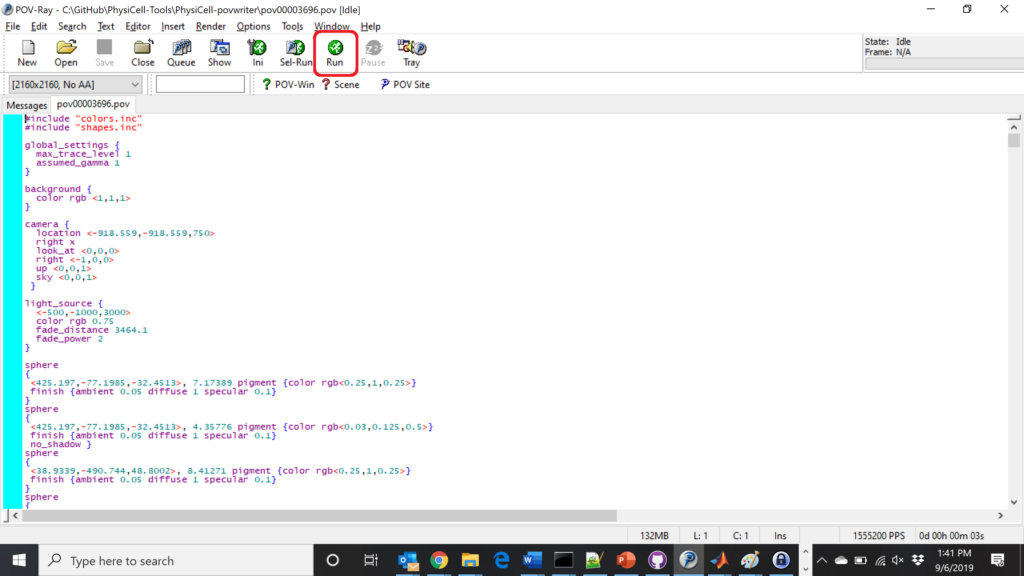You can watch the image as it renders. The result should be a PNG file (named pov00003696.png) that looks like this:

#### Using command-line options to process multiple times (option #1)

Now, suppose we have more outputs to process. We still state most of the options in the XML file as above, but now we also supply a command-line argument in the form of start:interval:end. If you’re still in the povwriter project, note that we have some more sample data there. Let’s grab and process it:

physicell$cd output physicell$ unzip more_samples.zip
Archive: more_samples.zip
inflating: output00000000_cells_physicell.mat
inflating: output00000001_cells_physicell.mat
inflating: output00000250_cells_physicell.mat
inflating: output00000300_cells_physicell.mat
inflating: output00000500_cells_physicell.mat
inflating: output00000750_cells_physicell.mat
inflating: output00001000_cells_physicell.mat
inflating: output00001250_cells_physicell.mat
inflating: output00001500_cells_physicell.mat
inflating: output00001750_cells_physicell.mat
inflating: output00002000_cells_physicell.mat
inflating: output00002250_cells_physicell.mat
inflating: output00002500_cells_physicell.mat
inflating: output00002750_cells_physicell.mat
inflating: output00003000_cells_physicell.mat
inflating: output00003250_cells_physicell.mat
inflating: output00003500_cells_physicell.mat
inflating: output00003696_cells_physicell.mat

physicell$ls citation and license.txt more_samples.zip output00000000_cells_physicell.mat output00000001_cells_physicell.mat output00000250_cells_physicell.mat output00000300_cells_physicell.mat output00000500_cells_physicell.mat output00000750_cells_physicell.mat output00001000_cells_physicell.mat output00001250_cells_physicell.mat output00001500_cells_physicell.mat output00001750_cells_physicell.mat output00002000_cells_physicell.mat output00002250_cells_physicell.mat output00002500_cells_physicell.mat output00002750_cells_physicell.mat output00003000_cells_physicell.mat output00003250_cells_physicell.mat output00003500_cells_physicell.mat output00003696.xml output00003696_cells_physicell.mat  Let’s go back to the parent directory and run povwriter: physicell$ ./povwriter 0:250:3500

povwriter version 1.0.0
================================================================================

Copyright (c) Paul Macklin 2019, on behalf of the PhysiCell project

Usage:
================================================================================
povwriter : run povwriter with config file ./config/settings.xml

povwriter FILENAME.xml : run povwriter with config file FILENAME.xml

povwriter x:y:z : run povwriter on data in FOLDER with indices from x
to y in incremenets of z

Example: ./povwriter 0:2:10 processes files:
./FOLDER/FILEBASE00000000_physicell_cells.mat
./FOLDER/FILEBASE00000002_physicell_cells.mat
...
./FOLDER/FILEBASE00000010_physicell_cells.mat
(See the config file to set FOLDER and FILEBASE)

povwriter x1,...,xn : run povwriter on data in FOLDER with indices x1,...,xn

Example: ./povwriter 1,3,17 processes files:
./FOLDER/FILEBASE00000001_physicell_cells.mat
./FOLDER/FILEBASE00000003_physicell_cells.mat
./FOLDER/FILEBASE00000017_physicell_cells.mat
(Note that there are no spaces.)
(See the config file to set FOLDER and FILEBASE)

Tutorial & documentation at http://MathCancer.org/blog/povwriter
================================================================================

Using config file ./config/povwriter-settings.xml ...
Using standard coloring function ...
Found 3 clipping planes ...
Found 2 cell color definitions ...
Matrix size: 32 x 18317
Processing file ./output/output00000000_cells_physicell.mat...
Creating file pov00000000.pov for output ...
Writing 18317 cells ...
Processing file ./output/output00002000_cells_physicell.mat...
Matrix size: 32 x 33551
Creating file pov00002000.pov for output ...
Writing 33551 cells ...
Processing file ./output/output00002500_cells_physicell.mat...
Matrix size: 32 x 43440
Creating file pov00002500.pov for output ...
Writing 43440 cells ...
Processing file ./output/output00001500_cells_physicell.mat...
Matrix size: 32 x 40267
Creating file pov00001500.pov for output ...
Writing 40267 cells ...
Processing file ./output/output00003000_cells_physicell.mat...
Matrix size: 32 x 56659
Creating file pov00003000.pov for output ...
Writing 56659 cells ...
Processing file ./output/output00001000_cells_physicell.mat...
Matrix size: 32 x 74057
Creating file pov00001000.pov for output ...
Writing 74057 cells ...
Processing file ./output/output00003500_cells_physicell.mat...
Matrix size: 32 x 66791
Creating file pov00003500.pov for output ...
Writing 66791 cells ...
Processing file ./output/output00000500_cells_physicell.mat...
Matrix size: 32 x 114316
Creating file pov00000500.pov for output ...
Writing 114316 cells ...
done!

Processing file ./output/output00000250_cells_physicell.mat...
Matrix size: 32 x 75352
Creating file pov00000250.pov for output ...
Writing 75352 cells ...
done!

Processing file ./output/output00002250_cells_physicell.mat...
Matrix size: 32 x 37959
Creating file pov00002250.pov for output ...
Writing 37959 cells ...
done!

Processing file ./output/output00001750_cells_physicell.mat...
Matrix size: 32 x 32358
Creating file pov00001750.pov for output ...
Writing 32358 cells ...
done!

Processing file ./output/output00002750_cells_physicell.mat...
Matrix size: 32 x 49658
Creating file pov00002750.pov for output ...
Writing 49658 cells ...
done!

Processing file ./output/output00003250_cells_physicell.mat...
Matrix size: 32 x 63546
Creating file pov00003250.pov for output ...
Writing 63546 cells ...
done!

done!

done!

done!

Processing file ./output/output00001250_cells_physicell.mat...
Matrix size: 32 x 54771
Creating file pov00001250.pov for output ...
Writing 54771 cells ...
done!

done!

done!

done!

Processing file ./output/output00000750_cells_physicell.mat...
Matrix size: 32 x 97642
Creating file pov00000750.pov for output ...
Writing 97642 cells ...
done!

done!

Done processing all 15 files!


Notice that the output appears a bit out of order. This is normal: povwriter is using 8 threads to process 8 files at the same time, and sending some output to the single screen. Since this is all happening simultaneously, it’s a bit jumbled (and non-sequential). Don’t panic. You should now have created pov00000000.povpov00000250.pov, … , pov00003500.pov.

Now, go into POV-ray, and choose “queue.” Click “Add File” and select all 15 .pov files you just created: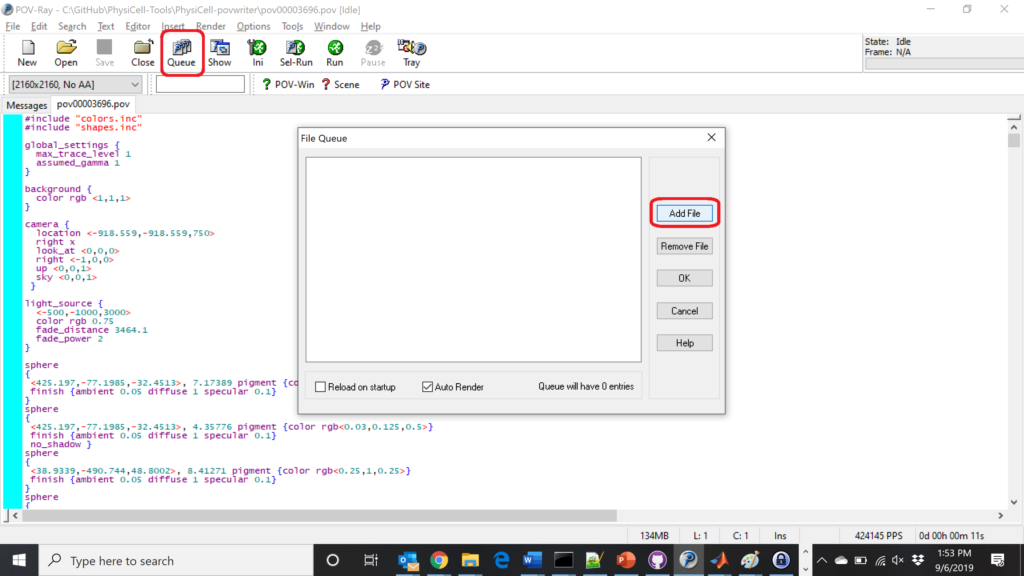Hit “OK” to let it render all the povray files to create PNG files (pov00000000.png, … , pov00003500.png).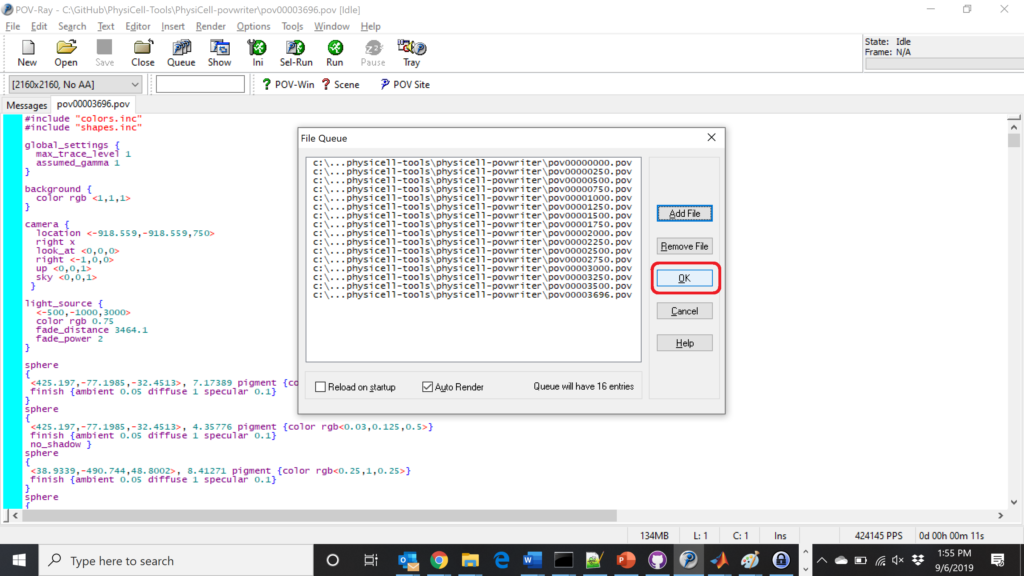#### Using command-line options to process multiple times (option #2)

You can also give a list of indices. Here’s how we render time indices 250, 1000, and 2250:



#### Creating an animated GIF with ImageMagick

Suppose you want to create an animated GIF based on your images. I suggest first converting to JPG (see above) and then using ImageMagick again. Here, I’m adding a 20 ms delay between frames:

physicell$magick convert -delay 20 *.jpg out.gif  Here’s the result: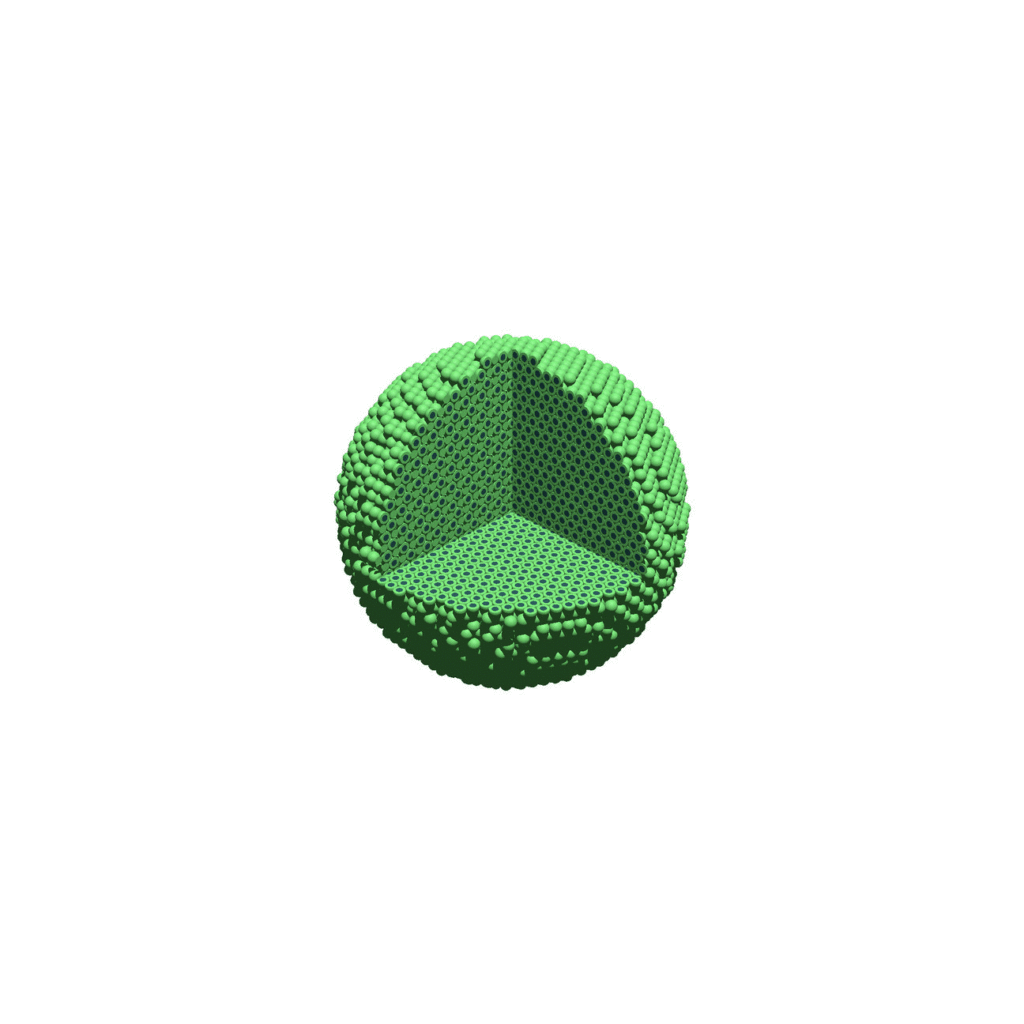Animated GIF created from raytraced still images. (You have to click the image to see the animation.) #### Creating a compressed movie with Mencoder Syntax coming later. ### Closing thoughts and future work In the future, we will probably allow more control over the clipping planes and a bit more debugging on how to handle planes that don’t pass through the origin. (First thoughts: we need to change how we use union and intersection commands in the POV-ray outputs.) We should also look at adding some transparency for the cells. I’d prefer something like rgba (red-green-blue-alpha), but POV-ray uses filters and transmission, and we want to make sure to get it right. Lastly, it would be nice to find a balance between the current very simple camera setup and better control. Thanks for reading this PhysiCell Friday tutorial! Please do give PhysiCell at try (at http://PhysiCell.org) and read the method paper at PLoS Computational Biology. Share this: Tags : ## User parameters in PhysiCell As of release 1.4.0, users can add any number of Boolean, integer, double, and string parameters to an XML configuration file. (These are stored by default in ./config/. The default parameter file is ./config/PhysiCell_settings.xml.) These parameters are automatically parsed into a parameters data structure, and accessible throughout a PhysiCell project. This tutorial will show you the key techniques to use these features. (See the User_Guide for full documentation.) First, let’s create a barebones 2D project by populating the 2D template project. In a terminal shell in your root PhysiCell directory, do this: make template2D  We will use this 2D project template for the remainder of the tutorial. We assume you already have a working copy of PhysiCell installed, version 1.4.0 or later. (If not, visit the PhysiCell tutorials to find installation instructions for your operating system.) ### User parameters in the XML configuration file Next, let’s look at the parameter file. In your text editor of choice, open up ./config/PhysiCell_settings.xml, and browse down to <user_parameters>, which will have some sample parameters from the 2D template project.  <user_parameters> <random_seed type="int" units="dimensionless">0</random_seed> <!-- example parameters from the template --> <!-- motile cell type parameters --> <motile_cell_persistence_time type="double" units="min">15</motile_cell_persistence_time> <motile_cell_migration_speed type="double" units="micron/min">0.5</motile_cell_migration_speed> <motile_cell_relative_adhesion type="double" units="dimensionless">0.05</motile_cell_relative_adhesion> <motile_cell_apoptosis_rate type="double" units="1/min">0.0</motile_cell_apoptosis_rate> <motile_cell_relative_cycle_entry_rate type="double" units="dimensionless">0.1</motile_cell_relative_cycle_entry_rate> </user_parameters>  Notice a few trends: • Each XML element (tag) under <user_parameters> is a user parameter, whose name is the element name. • Each variable requires an attribute named “type”, with one of the following four values: • bool for a Boolean parameter • int for an integer parameter • double for a double (floating point) parameter • string for text string parameter While we do not encourage it, if no valid type is supplied, PhysiCell will attempt to interpret the parameter as a double. • Each variable here has an (optional) attribute “units”. PhysiCell does not convert units, but these are helpful for clarity between users and developers. By default, PhysiCell uses minutes for all time units, and microns for all spatial units. • Then, between the tags, you list the value of your parameter. Let’s add the following parameters to the configuration file: • A string parameter called motile_color that sets the color of the motile_cell type in SVG outputs. Please refer to the User Guide (in the documentation folder) for more information on allowed color formats, including rgb values and named colors. Let’s use the value darkorange. • A double parameter called base_cycle_entry_rate that will give the rate of entry to the S cycle phase from the G1 phase for the default cell type in the code. Let’s use a ridiculously high value of 0.01 min-1. • A double parameter called base_apoptosis_rate for the default cell type. Let’s set the value at 1e-7 min-1. • A double parameter that sets the (relative) maximum cell-cell adhesion sensing distance, relative to the cell’s radius. Let’s set it at 2.5 (dimensionless). (The default is 1.25.) • A bool parameter that enables or disables placing a single motile cell in the initial setup. Let’s set it at true. If you edit the <user_parameters> to include these, it should look like this:  <user_parameters> <random_seed type="int" units="dimensionless">0</random_seed> <!-- example parameters from the template --> <!-- motile cell type parameters --> <motile_cell_persistence_time type="double" units="min">15</motile_cell_persistence_time> <motile_cell_migration_speed type="double" units="micron/min">0.5</motile_cell_migration_speed> <motile_cell_relative_adhesion type="double" units="dimensionless">0.05</motile_cell_relative_adhesion> <motile_cell_apoptosis_rate type="double" units="1/min">0.0</motile_cell_apoptosis_rate> <motile_cell_relative_cycle_entry_rate type="double" units="dimensionless">0.1</motile_cell_relative_cycle_entry_rate> <!-- for the tutorial --> <motile_color type="string" units="dimensionless">darkorange</motile_color> <base_cycle_entry_rate type="double" units="1/min">0.01</base_cycle_entry_rate> <base_apoptosis_rate type="double" units="1/min">1e-7</base_apoptosis_rate> <base_cell_adhesion_distance type="double" units="dimensionless">2.5</base_cell_adhesion_distance> <include_motile_cell type="bool" units="dimensionless">true</include_motile_cell> </user_parameters>  ### Viewing the loaded parameters Let’s compile and run the project. make ./project2D  At the beginning of the simulation, PhysiCell parses the <user_parameters> block into a global data structure called parameters, with sub-parts bools, ints, doubles, and strings. It displays these loaded parameters at the start of the simulation. Here’s what it looks like: shell$  ./project2D
Using config file ./config/PhysiCell_settings.xml ...
User parameters in XML config file:
Bool parameters::
include_motile_cell: 1 [dimensionless]

Int parameters::
random_seed: 0 [dimensionless]

Double parameters::
motile_cell_persistence_time: 15 [min]
motile_cell_migration_speed: 0.5 [micron/min]
motile_cell_apoptosis_rate: 0 [1/min]
motile_cell_relative_cycle_entry_rate: 0.1 [dimensionless]
base_cycle_entry_rate: 0.01 [1/min]
base_apoptosis_rate: 1e-007 [1/min]

String parameters::
motile_color: darkorange [dimensionless]


### Getting parameter values

Within a PhysiCell project, you can access the value of any parameter by either its index or its name, so long as you know its type. Here’s an example of accessing the base_cell_adhesion_distance by its name:

/* this directly accesses the value of the parameter */
double temp = parameters.doubles( "base_cell_adhesion_distance" );
std::cout << temp << std::endl;

/* this streams a formatted output including the parameter name and units */
std::cout << parameters.doubles[ "base_cell_adhesion_distance" ] << std::endl;

std::cout << parameters.doubles["base_cell_adhesion_distance"].name << " "


Notice that accessing by () gets the value of the parameter in a user-friendly way, whereas accessing by [] gets the entire parameter, including its name, value, and units.

You can more efficiently access the parameter by first finding its integer index, and accessing by index:

/* this directly accesses the value of the parameter */
int my_index = parameters.doubles.find_index( "base_cell_adhesion_distance" );
double temp = parameters.doubles( my_index );
std::cout << temp << std::endl;

/* this streams a formatted output including the parameter name and units */
std::cout << parameters.doubles[ my_index ] << std::endl;

std::cout << parameters.doubles[ my_index ].name << " "
<< parameters.doubles[ my_index ].value << " "
<< parameters.doubles[ my_index ].units << std::endl;


Similarly, we can access string and Boolean parameters. For example:

if( parameters.bools("include_motile_cell") == true )
{ std::cout << "I shall include a motile cell." << std::endl; }

int rand_ind = parameters.ints.find_index( "random_seed" );
std::cout << parameters.ints[rand_ind].name << " is at index " << rand_ind << std::endl;

std::cout << "We'll use this nice color: " << parameters.strings( "motile_color" );


### Using the parameters in custom functions

Let’s use these new parameters when setting up the parameter values of the simulation. For this project, all custom code is in ./custom_modules/custom.cpp. Open that source file in your favorite text editor. Look for the function called “create_cell_types“. In the code snipped below, we access the parameter values to set the appropriate parameters in the default cell definition, rather than hard-coding them.

	// add custom data here, if any

/* for the tutorial */
cell_defaults.phenotype.cycle.data.transition_rate(G0G1_index,S_index) =
parameters.doubles("base_cycle_entry_rate");
cell_defaults.phenotype.death.rates[apoptosis_model_index] =
parameters.doubles("base_apoptosis_rate");



Next, let’s change the tissue setup (“setup_tissue“) to check our Boolean variable before placing the initial motile cell.

     // now create a motile cell
/*  remove this conditional for the normal project */
if( parameters.bools("include_motile_cell") == true )
{
pC = create_cell( motile_cell );
pC->assign_position( 15.0, -18.0, 0.0 );
}


Lastly, let’s make use of the string parameter to change the plotting. Search for my_coloring_function and edit the source file to use the new color:

	// if the cell is motile and not dead, paint it black

static std::string motile_color = parameters.strings( "motile_color" );  // tutorial

if( pCell->phenotype.death.dead == false && pCell->type == 1 )
{
output = motile_color;
output = motile_color;
}


Notice the static here: We intend to call this function many, many times. For performance reasons, we don’t want to declare a string, instantiate it with motile_color, pass it to parameters.strings(), and then deallocate it once done. Instead, we store the search statically within the function, so that all future function calls will have access to that search result.

And that’s it! Compile your code, and give it a go.

make
./project2D


This should create a lot of data in the ./output directory, including SVG files that color motile cells as darkorange, like this one below.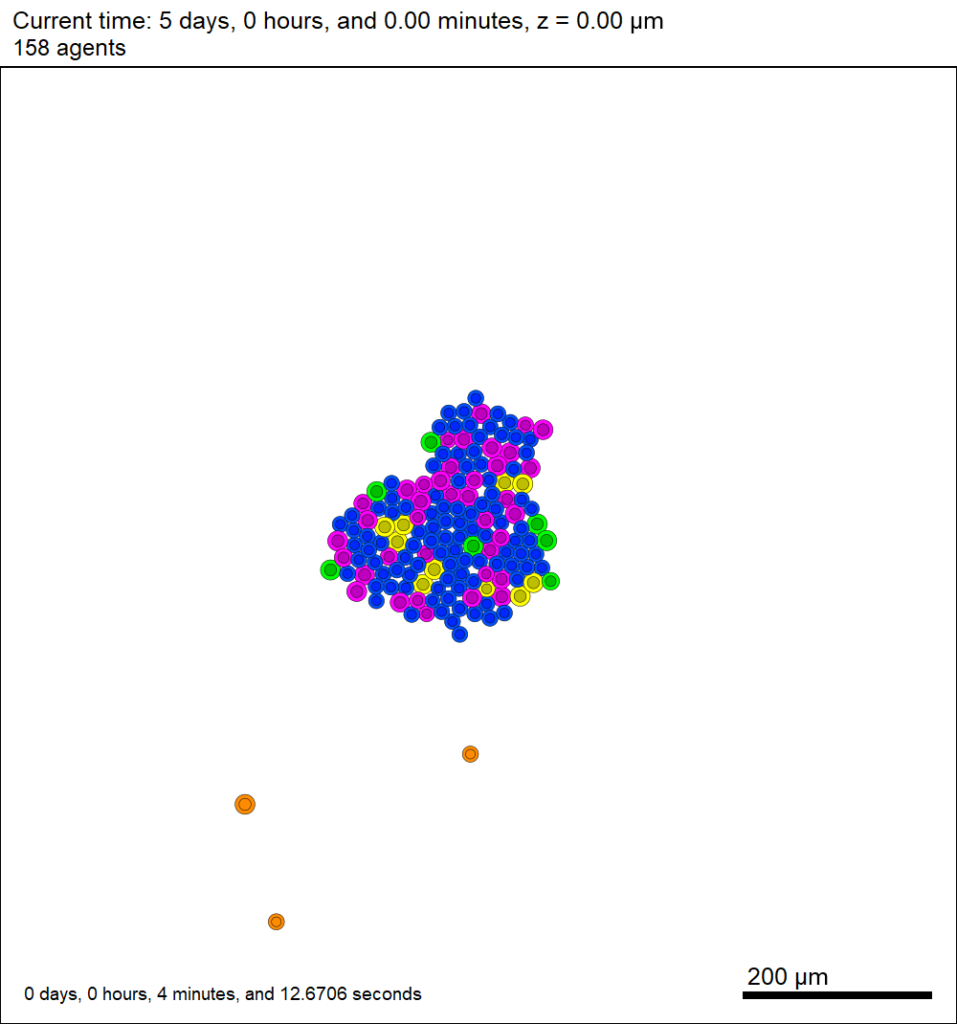Now that this project is parsing the XML file to get parameter values, we don’t need to recompile to change a model parameter. For example, change motile_color to mediumpurple, set motile_cell_migration_speed to 0.25, and set motile_cell_relative_cycle_entry_rate to 2.0. Rerun the code (without compiling):

./project2D


And let’s look at the change in the final SVG output (output00000120.svg):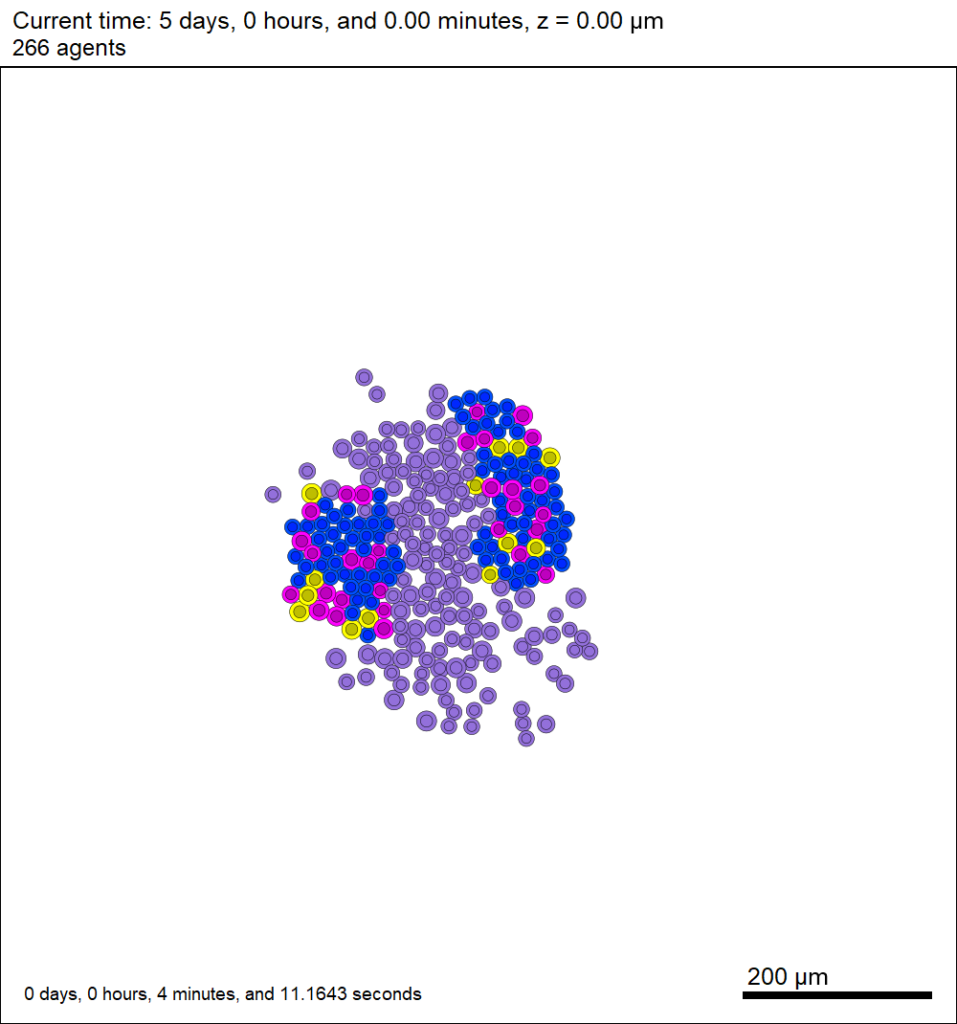### More notes on configuration files

You may notice other sections in the XML configuration file. I encourage you to explore them, but the meanings should be evident: you can set the computational domain size, the number of threads (for OpenMP parallelization), and how frequently (and where) data are stored. In future PhysiCell releases, we will continue adding more and more options to these XML files to simplify setup and configuration of PhysiCell models.

When you’re setting your BioFVM / PhysiCell g++ development environment, you’ll need to add the compiler, MSYS, and your text editor (like Notepad++) to your system path. For example, you may need to add folders like these to your system PATH variable:

1. c:\Program Files\mingw-w64\x86_64-5.3.0-win32-seh-rt_v4_rev0\mingw64\bin\
3. C:\MinGW\msys\1.0\bin\

Here’s how to do that in various versions of Windows.

### Windows XP, 7, and 8

First, open up a text editor, and concatenate your three paths into a single block of text, separated by semicolons (;):

2. Type a semicolon, paste in the first path, and append a semicolon. It should look like this:
;c:\Program Files\mingw-w64\x86_64-5.3.0-win32-seh-rt_v4_rev0\mingw64\bin\;
3. Paste in the next path, and append a semicolon. It should look like this:
;c:\Program Files\mingw-w64\x86_64-5.3.0-win32-seh-rt_v4_rev0\mingw64\bin\;C:\Program Files (x86)\Notepad++\;
4. Paste in the last path, and append a semicolon. It should look something like this:
;c:\Program Files\mingw-w64\x86_64-5.3.0-win32-seh-rt_v4_rev0\mingw64\bin\;C:\Program Files (x86)\Notepad++\;c:\MinGW\msys\1.0\bin\;

Lastly, add these paths to the system path:

1. Go the Start Menu, the right-click “This PC” or “My Computer”, and choose “Properties.”
2. Click on “Advanced system settings”
3. Click on “Environment Variables…” in the “Advanced” tab
4. Scroll through the “System Variables” below until you find Path.
5. Select “Path”, then click “Edit…”
6. At the very end of “Variable Value”, paste what you made in Notepad in the prior steps. Make sure to paste at the end of the existing value, rather than overwriting it!
7. Hit OK, OK, and OK to completely exit the “Advanced system settings.”

### Windows 10:

Windows 10 has made it harder to find these settings, but easier to edit them. First, let’s find the system path:

1. At the “run / search / Cortana” box next to the start menu, type “view advanced”, and you should see “view advanced system settings” auto-complete: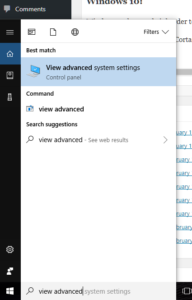2. Click to enter the advanced system settings, then choose environment variables … at the bottom of this box, and scroll down the list of user variables to Path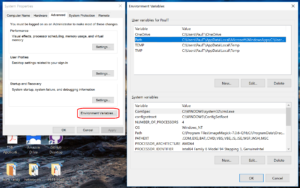3. Click on edit, then click New to add a new path. In the new entry (a new line), paste in your first new path (the compiler):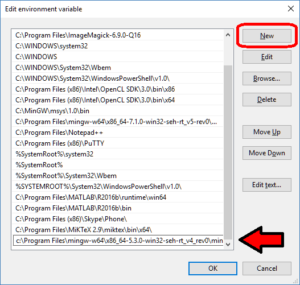4. Repeat this for the other two paths, then click OK, OK, Apply, OK to apply the new paths and exit.

## Working with PhysiCell MultiCellDS digital snapshots in MatlabPhysiCell 1.2.1 and later saves data as a specialized MultiCellDS digital snapshot, which includes chemical substrate fields, mesh information, and a readout of the cells and their phenotypes at single simulation time point. This tutorial will help you learn to use the matlab processing files included with PhysiCell.

This tutorial assumes you know (1) how to work at the shell / command line of your operating system, and (2) basic plotting and other functions in Matlab.

### Key elements of a PhysiCell digital snapshot

A PhysiCell digital snapshot (a customized form of the MultiCellDS digital simulation snapshot) includes the following elements saved as XML and MAT files:

1. output12345678.xml : This is the “base” output file, in MultiCellDS format. It includes key metadata such as when the file was created, the software, microenvironment information, and custom data saved at the simulation time. The Matlab files read this base file to find other related files (listed next). Example: output00003696.xml
2. initial_mesh0.mat : This is the computational mesh information for BioFVM at time 0.0. Because BioFVM and PhysiCell do not use moving meshes, we do not save this data at any subsequent time.
3. output12345678_microenvironment0.mat : This saves each biochemical substrate in the microenvironment at the computational voxels defined in the mesh (see above). Example: output00003696_microenvironment0.mat
4. output12345678_cells.mat : This saves very basic cellular information related to BioFVM, including cell positions, volumes, secretion rates, uptake rates, and secretion saturation densities. Example: output00003696_cells.mat
5. output12345678_cells_physicell.mat : This saves extra PhysiCell data for each cell agent, including volume information, cell cycle status, motility information, cell death information, basic mechanics, and any user-defined custom data. Example: output00003696_cells_physicell.mat

These snapshots make extensive use of Matlab Level 4 .mat files, for fast, compact, and well-supported saving of array data. Note that even if you cannot ready MultiCellDS XML files, you can work to parse the .mat files themselves.

### The PhysiCell Matlab .m files

Every PhysiCell distribution includes some matlab functions to work with PhysiCell digital simulation snapshots, stored in the matlab subdirectory. The main ones are:

1. composite_cutaway_plot.m : provides a quick, coarse 3-D cutaway plot of the discrete cells, with different colors for live (red), apoptotic (b), and necrotic (black) cells.
2. read_MultiCellDS_xml.m : reads the “base” PhysiCell snapshot and its associated matlab files.
3. set_MCDS_constants.m : creates a data structure MCDS_constants that has the same constants as PhysiCell_constants.h. This is useful for identifying cell cycle phases, etc.
4. simple_cutaway_plot.m : provides a quick, coarse 3-D cutaway plot of user-specified cells.
5. simple_plot.m : provides, a quick, coarse 3-D plot of the user-specified cells, without a cutaway or cross-sectional clipping plane.

#### A note on GNU Octave

Unfortunately, GNU octave does not include XML file parsing without some significant user tinkering. And one you’re done, it is approximately one order of magnitude slower than Matlab. Octave users can directly import the .mat files described above, but without the helpful metadata in the XML file. We’ll provide more information on the structure of these MAT files in a future blog post. Moreover, we plan to provide python and other tools for users without access to Matlab.

### A sample digital snapshot

We provide a 3-D simulation snapshot from the final simulation time of the cancer-immune example in Ghaffarizadeh et al. (2017, in review) at:

The corresponding SVG cross-section for that time (through = 0 μm) looks like this: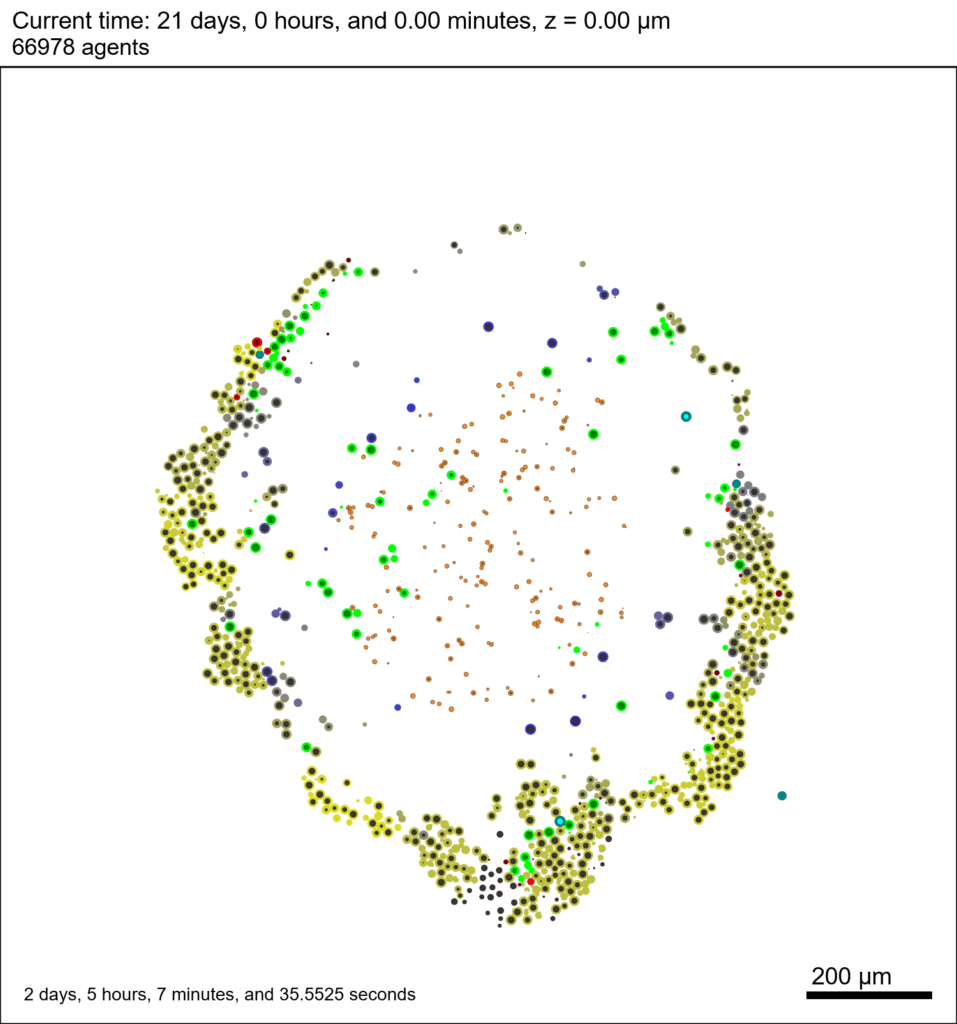Unzip the sample dataset in any directory, and make sure the matlab files above are in the same directory (or in your Matlab path). If you’re inside matlab:

!unzip 3D_PhysiCell_matlab_sample.zip


MCDS = read_MultiCellDS_xml( 'output00003696.xml');


This will load the mesh, substrates, and discrete cells into the MCDS data structure, and give a basic summary: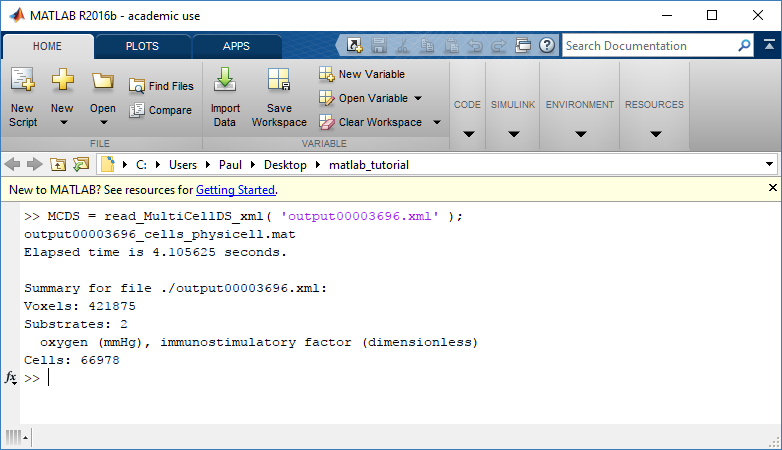Typing ‘MCDS’ and then hitting ‘tab’ (for auto-completion) shows the overall structure of MCDS, stored as metadata, mesh, continuum variables, and discrete cells: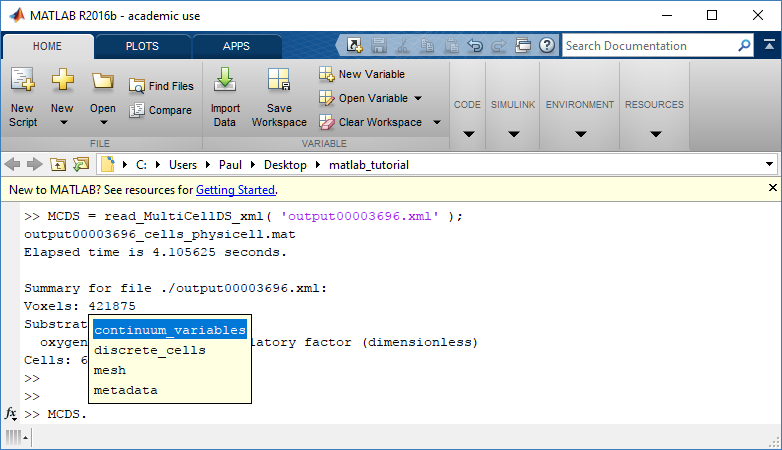To get simulation metadata, such as the current simulation time, look at MCDS.metadata.current_time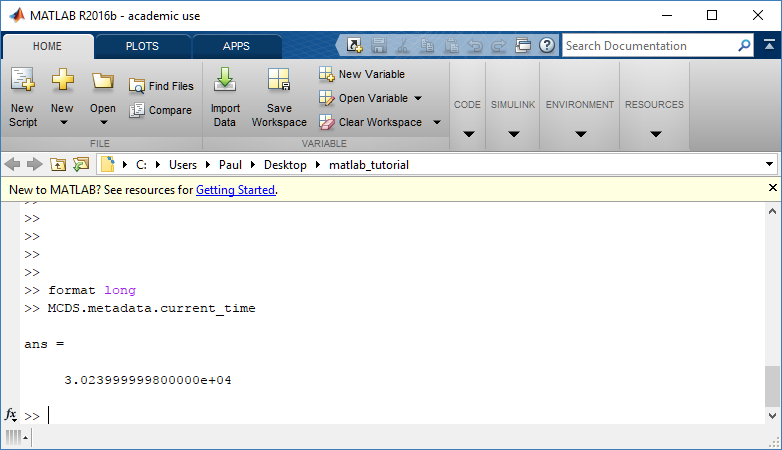Here, we see that the current simulation time is 30240 minutes, or 21 days. MCDS.metadata.current_runtime gives the elapsed walltime to up to this point: about 53 hours (1.9e5 seconds), including file I/O time to write full simulation data once per 3 simulated minutes after the start of the adaptive immune response.

### Plotting chemical substrates

Let’s make an oxygen contour plot through z = 0 μm. First, we find the index corresponding to this z-value:

k = find( MCDS.mesh.Z_coordinates == 0 );


Next, let’s figure out which variable is oxygen. Type “MCDS.continuum_variables.name”, which will show the array of variable names: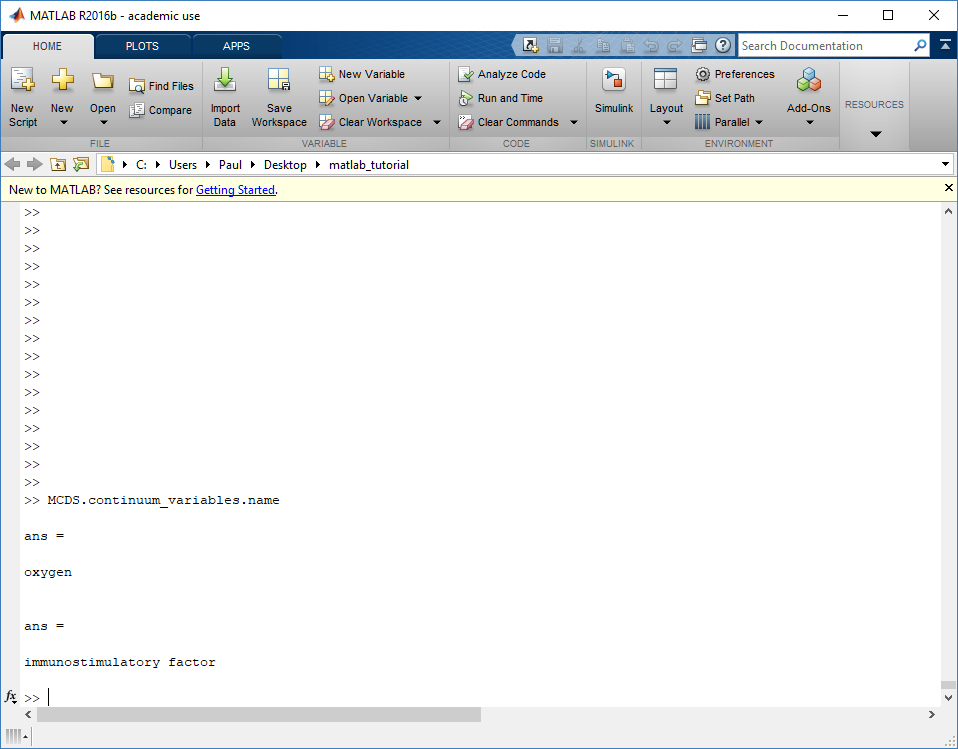Here, oxygen is the first variable, (index 1). So, to make a filled contour plot:

contourf( MCDS.mesh.X(:,:,k), MCDS.mesh.Y(:,:,k), ...
MCDS.continuum_variables(1).data(:,:,k) , 20 ) ;


Now, let’s set this to a correct aspect ratio (no stretching in x or y), add a colorbar, and set the axis labels, using

axis image
colorbar
xlabel( sprintf( 'x (%s)' , MCDS.metadata.spatial_units) );
ylabel( sprintf( 'y (%s)' , MCDS.metadata.spatial_units) );


Lastly, let’s add an appropriate (time-based) title:

title( sprintf('%s (%s) at t = %3.2f %s, z = %3.2f %s', MCDS.continuum_variables(1).name , ...
MCDS.continuum_variables(1).units , ...
MCDS.mesh.Z_coordinates(k), ...


Here’s the end result: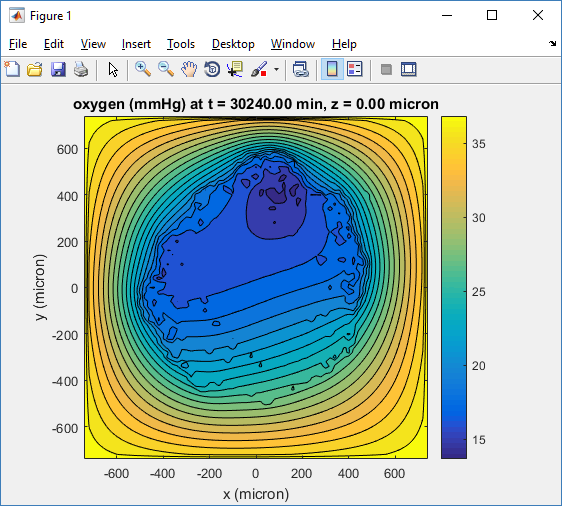We can easily export graphics, such as to PNG format:

print( '-dpng' , 'output_o2.png' );


For more on plotting BioFVM data, see the tutorial
at http://www.mathcancer.org/blog/saving-multicellds-data-from-biofvm/

### Plotting cells in space

#### 3-D point cloud

First, let’s plot all the cells in 3D:

plot3( MCDS.discrete_cells.state.position(:,1) , MCDS.discrete_cells.state.position(:,2), ...
MCDS.discrete_cells.state.position(:,3) , 'bo' );


At first glance, this does not look good: some cells are far out of the simulation domain, distorting the automatic range of the plot:This does not ordinarily happen in PhysiCell (the default cell mechanics functions have checks to prevent such behavior), but this example includes a simple Hookean elastic adhesion model for immune cell attachment to tumor cells. In rare circumstances, an attached tumor cell or immune cell can apoptose on its own (due to its background apoptosis rate),
without “knowing” to detach itself from the surviving cell in the pair. The remaining cell attempts to calculate its elastic velocity based upon an invalid cell position (no longer in memory), creating an artificially large velocity that “flings” it out of the simulation domain. Such cells  are not simulated any further, so this is effectively equivalent to an extra apoptosis event (only 3 cells are out of the simulation domain after tens of millions of cell-cell elastic adhesion calculations). Future versions of this example will include extra checks to prevent this rare behavior.

The plot can simply be fixed by changing the axis:

axis( 1000*[-1 1 -1 1 -1 1] )
axis square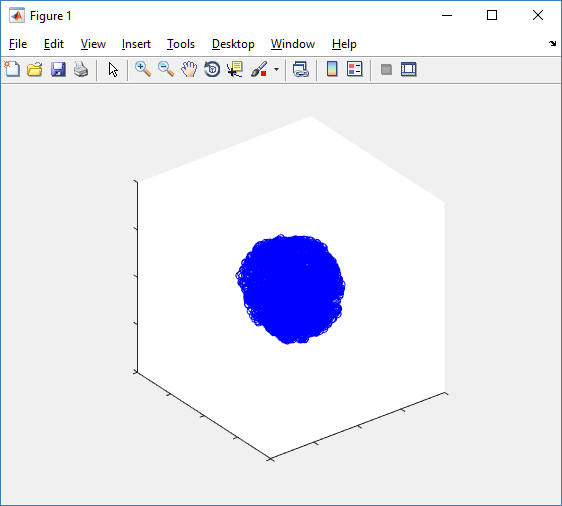Notice that this is a very difficult plot to read, and very non-interactive (laggy) to rotation and scaling operations. We can make a slightly nicer plot by searching for different cell types and plotting them with different colors:

% make it easier to work with the cell positions;
P = MCDS.discrete_cells.state.position;

% find type 1 cells
ind1 = find( MCDS.discrete_cells.metadata.type == 1 );
% better still, eliminate those out of the simulation domain
ind1 = find( MCDS.discrete_cells.metadata.type == 1 & ...
abs(P(:,1))' < 1000 & abs(P(:,2))' < 1000 & abs(P(:,3))' < 1000 );

% find type 0 cells
ind0 = find( MCDS.discrete_cells.metadata.type == 0 & ...
abs(P(:,1))' < 1000 & abs(P(:,2))' < 1000 & abs(P(:,3))' < 1000 );

%now plot them
P = MCDS.discrete_cells.state.position;
plot3( P(ind0,1), P(ind0,2), P(ind0,3), 'bo' )
hold on
plot3( P(ind1,1), P(ind1,2), P(ind1,3), 'ro' )
hold off
axis( 1000*[-1 1 -1 1 -1 1] )
axis square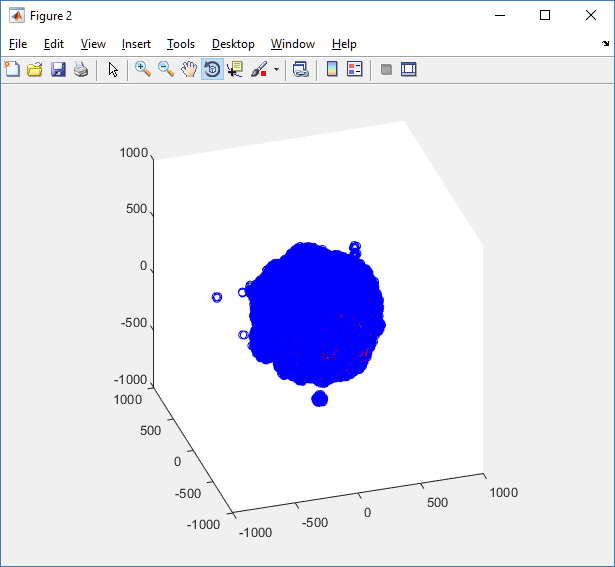However, this isn’t much better. You can use the scatter3 function to gain more control on the size and color of the plotted cells, or even make macros to plot spheres in the cell locations (with shading and lighting), but Matlab is very slow when plotting beyond 103 cells. Instead, we recommend the faster preview functions below for data exploration, and higher-quality plotting (e.g., by POV-ray) for final publication-

#### Fast 3-D cell data previewers

Notice that plot3 and scatter3 are painfully slow for any nontrivial number of cells. We can use a few fast previewers to quickly get a sense of the data. First, let’s plot all the dead cells, and make them red:

clf
simple_plot( MCDS,  MCDS, MCDS.discrete_cells.dead_cells , 'r' )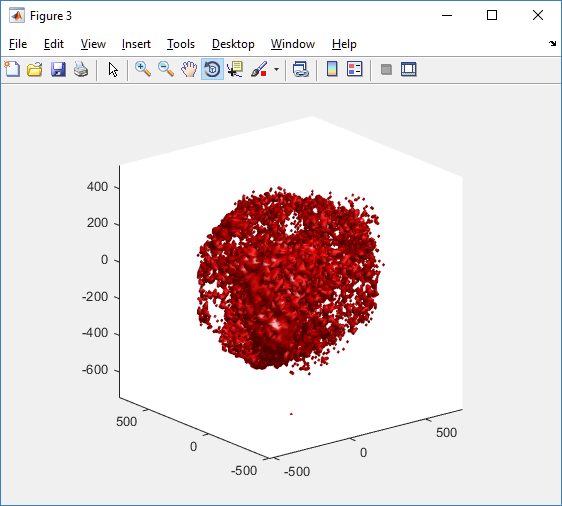This function creates a coarse-grained 3-D indicator function (0 if no cells are present; 1 if they are), and plots a 3-D level surface. It is very responsive to rotations and other operations to explore the data. You may notice the second argument is a list of indices: only these cells are plotted. This gives you a method to select cells with specific characteristics when plotting. (More on that below.) If you want to get a sense of the interior structure, use a cutaway plot:

clf
simple_cutaway_plot( MCDS, MCDS, MCDS.discrete_cells.dead_cells , 'r' )We also provide a fast “composite” cutaway which plots all live cells as red, apoptotic cells as blue (without the cutaway), and all necrotic cells as black:

clf
composite_cutaway_plot( MCDS )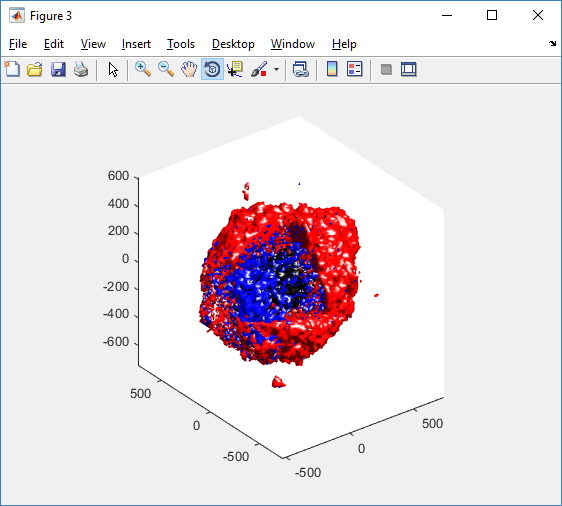Lastly, we show an improved plot that uses different colors for the immune cells, and Matlab’s “find” function to help set up the indexing:

constants = set_MCDS_constants

% find the type 0 necrotic cells
ind0_necrotic = find( MCDS.discrete_cells.metadata.type == 0 & ...
(MCDS.discrete_cells.phenotype.cycle.current_phase == constants.necrotic_swelling | ...
MCDS.discrete_cells.phenotype.cycle.current_phase == constants.necrotic_lysed | ...
MCDS.discrete_cells.phenotype.cycle.current_phase == constants.necrotic) );

% find the live type 0 cells
ind0_live = find( MCDS.discrete_cells.metadata.type == 0 & ...
(MCDS.discrete_cells.phenotype.cycle.current_phase ~= constants.necrotic_swelling & ...
MCDS.discrete_cells.phenotype.cycle.current_phase ~= constants.necrotic_lysed & ...
MCDS.discrete_cells.phenotype.cycle.current_phase ~= constants.necrotic & ...
MCDS.discrete_cells.phenotype.cycle.current_phase ~= constants.apoptotic) );

clf
% plot live tumor cells red, in cutaway view
simple_cutaway_plot( MCDS, ind0_live , 'r' );
hold on
% plot dead tumor cells black, in cutaway view
simple_cutaway_plot( MCDS, ind0_necrotic , 'k' )
% plot all immune cells, but without cutaway (to show how they infiltrate)
simple_plot( MCDS, ind1, 'g' )
hold off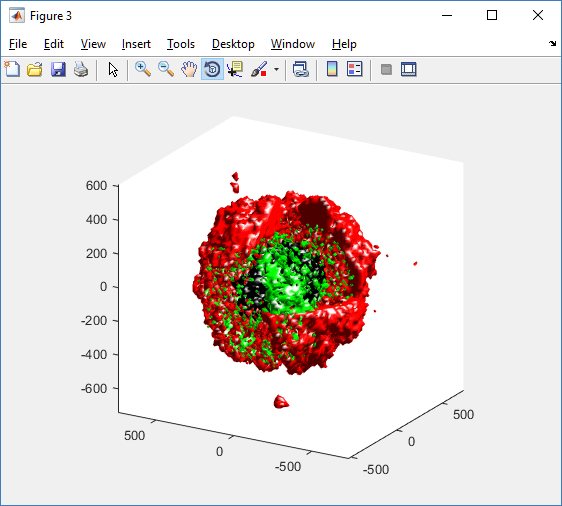### A small cautionary note on future compatibility

PhysiCell 1.2.1 uses the <custom> data tag (allowed as part of the MultiCellDS specification) to encode its cell data, to allow a more compact data representation, because the current PhysiCell daft does not support such a formulation, and Matlab is painfully slow at parsing XML files larger than ~50 MB. Thus, PhysiCell snapshots are not yet fully compatible with general MultiCellDS tools, which would by default ignore custom data. In the future, we will make available converter utilities to transform “native” custom PhysiCell snapshots to MultiCellDS snapshots that encode all the cellular information in a more verbose but compatible XML format.

### Closing words and future work

Because Octave is not a great option for parsing XML files (with critical MultiCellDS metadata), we plan to write similar functions to read and plot PhysiCell snapshots in Python, as an open source alternative. Moreover, our lab in the next year will focus on creating further MultiCellDS configuration, analysis, and visualization routines. We also plan to provide additional 3-D functions for plotting the discrete cells and varying color with their properties.

In the longer term, we will develop open source, stand-alone analysis and visualization tools for MultiCellDS snapshots (including PhysiCell snapshots). Please stay tuned!

Tags :

## Coarse-graining discrete cell cycle models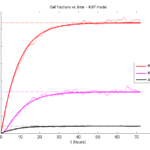### Introduction

One observation that often goes underappreciated in computational biology discussions is that a computational model is often a model of a model of a model of biology: that is, it’s a numerical approximation (a model) of a mathematical model of an experimental model of a real-life biological system. Thus, there are three big places where a computational investigation can fall flat:

1. The experimental model may be a bad choice for the disease or process (not our fault).
2. Second, the mathematical model of the experimental system may have flawed assumptions (something we have to evaluate).
3. The numerical implementation may have bugs or otherwise be mathematically inconsistent with the mathematical model.

Critically, you can’t use simulations to evaluate the experimental model or the mathematical model until you verify that the numerical implementation is consistent with the mathematical model, and that the numerical solution converges as $$\Delta t$$ and $$\Delta x$$ shrink to zero.

There are numerous ways to accomplish this, but ideally, it boils down to having some analytical solutions to the mathematical model, and comparing numerical solutions to these analytical or theoretical results. In this post, we’re going to walk through the math of analyzing a typical type of discrete cell cycle model.

### Discrete model

Suppose we have a cell cycle model consisting of phases $$P_1, P_2, \ldots P_n$$, where cells in the $$P_i$$ phase progress to the $$P_{i+1}$$ phase after a mean waiting time of $$T_i$$, and cells leaving the $$P_n$$ phase divide into two cells in the $$P_1$$ phase. Assign each cell agent $$k$$ a current phenotypic phase $$S_k(t)$$. Suppose also that each phase $$i$$ has a death rate $$d_i$$, and that cells persist for on average $$T_\mathrm{A}$$ time in the dead state before they are removed from the simulation.

The mean waiting times $$T_i$$ are equivalent to transition rates $$r_i = 1 / T_i$$ (Macklin et al. 2012). Moreover, for any time interval $$[t,t+\Delta t]$$, both are equivalent to a transition probability of
$\mathrm{Prob}\Bigl( S_k(t+\Delta t) = P_{i+1} | S(t) = P_i \Bigr) = 1 – e^{ -r_i \Delta t } \approx r_i \Delta t = \frac{ \Delta t}{ T_i}.$ In many discrete models (especially cellular automaton models) with fixed step sizes $$\Delta t$$, models are stated in terms of transition probabilities $$p_{i,i+1}$$, which we see are equivalent to the work above with $$p_{i,i+1} = r_i \Delta t = \Delta t / T_i$$, allowing us to tie mathematical model forms to biological, measurable parameters. We note that each $$T_i$$ is the average duration of the $$P_i$$ phase.

#### Concrete example: a Ki67 Model

Ki-67 is a nuclear protein that is expressed through much of the cell cycle, including S, G2, M, and part of G1 after division. It is used very commonly in pathology to assess proliferation, particularly in cancer. See the references and discussion in (Macklin et al. 2012). In Macklin et al. (2012), we came up with a discrete cell cycle model to match Ki-67 data (along with cleaved Caspase-3 stains for apoptotic cells). Let’s summarize the key parts here.

Each cell agent $$i$$ has a phase $$S_i(t)$$. Ki67- cells are quiescent (phase $$Q$$, mean duration $$T_\mathrm{Q}$$), and they can enter the Ki67+ $$K_1$$ phase (mean duration $$T_1$$). When $$K_1$$ cells leave their phase, they divide into two Ki67+ daughter cells in the $$K_2$$ phase with mean duration $$T_2$$. When cells exit $$K_2$$, they return to $$Q$$. Cells in any phase can become apoptotic (enter the $$A$$ phase with mean duration $$T_\mathrm{A}$$), with death rate $$r_\mathrm{A}$$.

### Coarse-graining to an ODE model

If each phase $$i$$ has a death rate $$d_i$$, if $$N_i(t)$$ denotes the number of cells in the $$P_i$$ phase at time $$t$$, and if $$A(t)$$ is the number of dead (apoptotic) cells at time $$t$$, then on average, the number of cells in the $$P_i$$ phase at the next time step is given by
$N_i(t+\Delta t) = N_i(t) + N_{i-1}(t) \cdot \left[ \textrm{prob. of } P_{i-1} \rightarrow P_i \textrm{ transition} \right] – N_i(t) \cdot \left[ \textrm{prob. of } P_{i} \rightarrow P_{i+1} \textrm{ transition} \right]$ $– N_i(t) \cdot \left[ \textrm{probability of death} \right]$ By the work above, this is:
$N_i(t+\Delta t) \approx N_i(t) + N_{i-1}(t) r_{i-1} \Delta t – N_i(t) r_i \Delta t – N_i(t) d_i \Delta t ,$ or after shuffling terms and taking the limit as $$\Delta t \downarrow 0$$, $\frac{d}{dt} N_i(t) = r_{i-1} N_{i-1}(t) – \left( r_i + d_i \right) N_i(t).$ Continuing this analysis, we obtain a linear system:
$\frac{d}{dt}{ \vec{N} } = \begin{bmatrix} -(r_1+d_1) & 0 & \cdots & 0 & 2r_n & 0 \\ r_1 & -(r_2+d_2) & 0 & \cdots & 0 & 0 \\ 0 & r_2 & -(r_3+d_3) & 0 & \cdots & 0 \\ & & \ddots & & \\0&\cdots&0 &r_{n-1} & -(r_n+d_n) & 0 \\ d_1 & d_2 & \cdots & d_{n-1} & d_n & -\frac{1}{T_\mathrm{A}} \end{bmatrix}\vec{N} = M \vec{N},$ where $$\vec{N}(t) = [ N_1(t), N_2(t) , \ldots , N_n(t) , A(t) ]$$.

For the Ki67 model above, let $$\vec{N} = [K_1, K_2, Q, A]$$. Then the linear system is
$\frac{d}{dt} \vec{N} = \begin{bmatrix} -\left( \frac{1}{T_1} + r_\mathrm{A} \right) & 0 & \frac{1}{T_\mathrm{Q}} & 0 \\ \frac{2}{T_1} & -\left( \frac{1}{T_2} + r_\mathrm{A} \right) & 0 & 0 \\ 0 & \frac{1}{T_2} & -\left( \frac{1}{T_\mathrm{Q}} + r_\mathrm{A} \right) & 0 \\ r_\mathrm{A} & r_\mathrm{A} & r_\mathrm{A} & -\frac{1}{T_\mathrm{A}} \end{bmatrix} \vec{N} .$
(If we had written $$\vec{N} = [Q, K_1, K_2 , A]$$, then the matrix above would have matched the general form.)

### Some theoretical results

If $$M$$ has eigenvalues $$\lambda_1 , \ldots \lambda_{n+1}$$ and corresponding eigenvectors $$\vec{v}_1, \ldots , \vec{v}_{n+1}$$, then the general solution is given by
$\vec{N}(t) = \sum_{i=1}^{n+1} c_i e^{ \lambda_i t } \vec{v}_i ,$ and if the initial cell counts are given by $$\vec{N}(0)$$ and we write $$\vec{c} = [c_1, \ldots c_{n+1} ]$$, we can obtain the coefficients by solving $\vec{N}(0) = [ \vec{v}_1 | \cdots | \vec{v}_{n+1} ]\vec{c} .$ In many cases, it turns out that all but one of the eigenvalues (say $$\lambda$$ with corresponding eigenvector $$\vec{v}$$) are negative. In this case, all the other components of the solution decay away, and for long times, we have $\vec{N}(t) \approx c e^{ \lambda t } \vec{v} .$ This is incredibly useful, because it says that over long times, the fraction of cells in the $$i^\textrm{th}$$ phase is given by $v_{i} / \sum_{j=1}^{n+1} v_{j}.$

#### Matlab implementation (with the Ki67 model)

First, let’s set some parameters, to make this a little easier and reusable.

parameters.dt = 0.1; % 6 min = 0.1 hours
parameters.time_units = 'hour';
parameters.t_max = 3*24; % 3 days

parameters.K1.duration =  13;
parameters.K1.death_rate = 1.05e-3;
parameters.K1.initial = 0;

parameters.K2.duration = 2.5;
parameters.K2.death_rate = 1.05e-3;
parameters.K2.initial = 0;

parameters.Q.duration = 74.35 ;
parameters.Q.death_rate = 1.05e-3;
parameters.Q.initial = 1000;

parameters.A.duration = 8.6;
parameters.A.initial = 0;


Next, we write a function to read in the parameter values, construct the matrix (and all the data structures), find eigenvalues and eigenvectors, and create the theoretical solution. It also finds the positive eigenvalue to determine the long-time values.

function solution = Ki67_exact( parameters )

% allocate memory for the main outputs

solution.T = 0:parameters.dt:parameters.t_max;
solution.K1 = zeros( 1 , length(solution.T));
solution.K2 = zeros( 1 , length(solution.T));
solution.K = zeros( 1 , length(solution.T));
solution.Q = zeros( 1 , length(solution.T));
solution.A = zeros( 1 , length(solution.T));
solution.Live = zeros( 1 , length(solution.T));
solution.Total = zeros( 1 , length(solution.T));

% allocate memory for cell fractions

solution.AI = zeros(1,length(solution.T));
solution.KI1 = zeros(1,length(solution.T));
solution.KI2 = zeros(1,length(solution.T));
solution.KI = zeros(1,length(solution.T));

% get the main parameters

T1 = parameters.K1.duration;
r1A = parameters.K1.death_rate;

T2 = parameters.K2.duration;
r2A = parameters.K2.death_rate;

TQ = parameters.Q.duration;
rQA = parameters.Q.death_rate;

TA = parameters.A.duration;

% write out the mathematical model:
% d[Populations]/dt = Operator*[Populations]

Operator = [ -(1/T1 +r1A) , 0 , 1/TQ , 0; ...
2/T1 , -(1/T2 + r2A) ,0 , 0; ...
0 , 1/T2 , -(1/TQ + rQA) , 0; ...
r1A , r2A, rQA , -1/TA ];

% eigenvectors and eigenvalues

[V,D] = eig(Operator);
eigenvalues = diag(D);

% save the eigenvectors and eigenvalues in case you want them.

solution.V = V;
solution.D = D;
solution.eigenvalues = eigenvalues;

% initial condition

VecNow = [ parameters.K1.initial ; parameters.K2.initial ; ...
parameters.Q.initial ; parameters.A.initial ] ;
solution.K1(1) = VecNow(1);
solution.K2(1) = VecNow(2);
solution.Q(1) = VecNow(3);
solution.A(1) = VecNow(4);
solution.K(1) = solution.K1(1) + solution.K2(1);
solution.Live(1) = sum( VecNow(1:3) );
solution.Total(1) = sum( VecNow(1:4) );

solution.AI(1) = solution.A(1) / solution.Total(1);
solution.KI1(1) = solution.K1(1) / solution.Total(1);
solution.KI2(1) = solution.K2(1) / solution.Total(1);
solution.KI(1) = solution.KI1(1) + solution.KI2(1);

% now, get the coefficients to write the analytic solution
% [Populations] = c1*V(:,1)*exp( d(1,1)*t) + c2*V(:,2)*exp( d(2,2)*t ) +
%                 c3*V(:,3)*exp( d(3,3)*t) + c4*V(:,4)*exp( d(4,4)*t );

coeff = linsolve( V , VecNow );

% find the (hopefully one) positive eigenvalue.
% eigensolutions with negative eigenvalues decay,
% leaving this as the long-time behavior.

eigenvalues = diag(D);
n = find( real( eigenvalues ) &amp;gt; 0 )
solution.long_time.KI1 = V(1,n) / sum( V(:,n) );
solution.long_time.KI2 = V(2,n) / sum( V(:,n) );
solution.long_time.QI = V(3,n) / sum( V(:,n) );
solution.long_time.AI = V(4,n) / sum( V(:,n) ) ;
solution.long_time.KI = solution.long_time.KI1 + solution.long_time.KI2;

% now, write out the solution at all the times
for i=2:length( solution.T )
% compact way to write the solution
VecExact = real( V*( coeff .* exp( eigenvalues*solution.T(i) ) ) );

solution.K1(i) = VecExact(1);
solution.K2(i) = VecExact(2);
solution.Q(i) = VecExact(3);
solution.A(i) = VecExact(4);
solution.K(i) = solution.K1(i) + solution.K2(i);
solution.Live(i) = sum( VecExact(1:3) );
solution.Total(i) = sum( VecExact(1:4) );

solution.AI(i) = solution.A(i) / solution.Total(i);
solution.KI1(i) = solution.K1(i) / solution.Total(i);
solution.KI2(i) = solution.K2(i) / solution.Total(i);
solution.KI(i) = solution.KI1(i) + solution.KI2(i);
end

return;


Now, let’s run it and see what this thing looks like: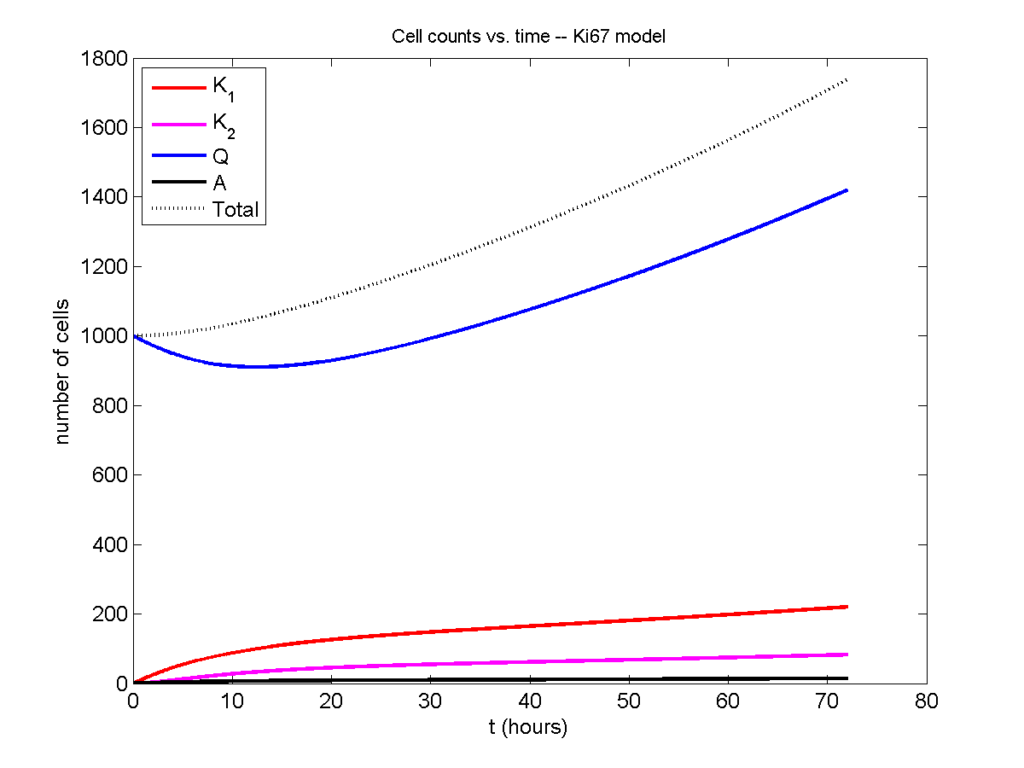Next, we plot KI1, KI2, and AI versus time (solid curves), along with the theoretical long-time behavior (dashed curves). Notice how well it matches–it’s neat when theory works! :-)Some readers may recognize the long-time fractions: KI1 + KI2 = KI = 0.1743, and AI = 0.00833, very close to the DCIS patient values from our simulation study in Macklin et al. (2012) and improved calibration work in Hyun and Macklin (2013).

### Comparing simulations and theory

I wrote a small Matlab program to implement the discrete model: start with 1000 cells in the $$Q$$ phase, and in each time interval $$[t,t+\Delta t]$$, each cell “decides” whether to advance to the next phase, stay in the same phase, or apoptose. If we compare a single run against the theoretical curves, we see hints of a match:

If we average 10 simulations and compare, the match is better:

And lastly, if we average 100 simulations and compare, the curves are very difficult to tell apart:

Even in logarithmic space, it’s tough to tell these apart: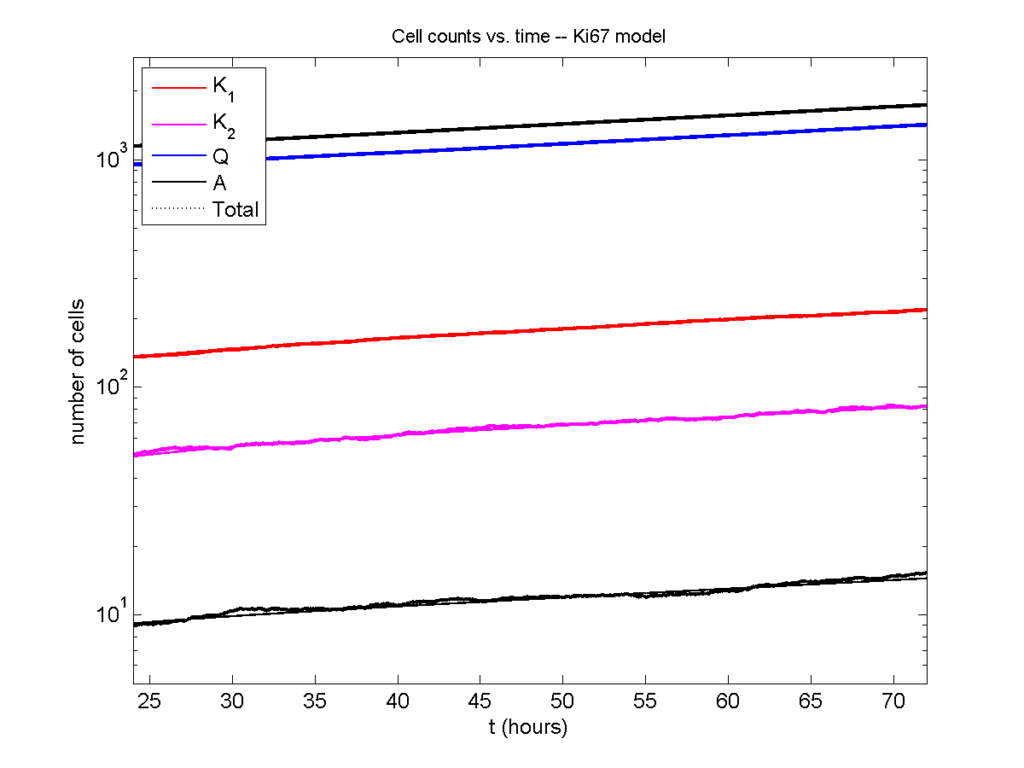### Code

The following matlab files (available here) can be used to reproduce this post:

Ki67_exact.m
The function defined above to create the exact solution using the eigenvalue/eignvector approach.
Ki67_stochastic.m
Runs a single stochastic simulation, using the supplied parameters.
script.m
Runs the theoretical solution first, creates plots, and then runs the stochastic model 100 times for comparison.

To make it all work, simply run “script” at the command prompt. Please note that it will generate some png files in its directory.

### Closing thoughts

In this post, we showed a nice way to check a discrete model against theoretical behavior–both in short-term dynamics and long-time behavior. The same work should apply to validating many discrete models. However, when you add spatial effects (e.g., a cellular automaton model that won’t proliferate without an empty neighbor site), I wouldn’t expect a match. (But simulating cells that initially have a “salt and pepper”, random distribution should match this for early times.)

Moreover, models with deterministic phase durations (e.g., K1, K2, and A have fixed durations) aren’t consistent with the ODE model above, unless the cells they are each initialized with a random amount of “progress” in their initial phases. (Otherwise, the cells in each phase will run synchronized, and there will be fixed delays before cells transition to other phases.) Delay differential equations better describe such models. However, for long simulation times, the slopes of the sub-populations and the cell fractions should start to better and better match the ODE models.

Now that we have verified that the discrete model is performing as expected, we can have greater confidence in its predictions, and start using those predictions to assess the underlying models. In ODE and PDE models, you often validate the code on simpler problems where you have an analytical solution, and then move on to making simulation predictions in cases where you can’t solve analytically. Similarly, we can now move on to variants of the discrete model where we can’t as easily match ODE theory (e.g., time-varying rate parameters, spatial effects), but with the confidence that the phase transitions are working as they should.

## BioFVM warmup: 2D continuum simulation of tumor growth

Note: This is part of a series of “how-to” blog posts to help new users and developers of BioFVM. See also the guides to setting up a C++ compiler in Windows or OSX.

### What you’ll need

1. A working C++ development environment with support for OpenMP. See these prior tutorials if you need help.
2. A download of BioFVM, available at http://BioFVM.MathCancer.org and http://BioFVM.sf.net. Use Version 1.0.3 or later.
3. Matlab or Octave for visualization. Matlab might be available for free at your university. Octave is open source and available from a variety of sources.

We will implement a basic 2-D model of tumor growth in a heterogeneous microenvironment, with inspiration by glioblastoma models by Kristin Swanson, Russell Rockne and others (e.g., this work), and continuum tumor growth models by Hermann Frieboes, John Lowengrub, and our own lab (e.g., this paper and this paper).

We will model tumor growth driven by a growth substrate, where cells die when the growth substrate is insufficient. The tumor cells will have motility. A continuum blood vasculature will supply the growth substrate, but tumor cells can degrade this existing vasculature. We will revisit and extend this model from time to time in future tutorials.

### Mathematical model

Taking inspiration from the groups mentioned above, we’ll model a live cell density ρ of a relatively low-adhesion tumor cell species (e.g., glioblastoma multiforme). We’ll assume that tumor cells move randomly towards regions of low cell density (modeled as diffusion with motility μ). We’ll assume that that the net birth rate rB is proportional to the concentration of growth substrate σ, which is released by blood vasculature with density b. Tumor cells can degrade the tissue and hence this existing vasculature. Tumor cells die at rate rD when the growth substrate level is too low. We assume that the tumor cell density cannot exceed a max level ρmax. A model that includes these effects is:

$\frac{ \partial \rho}{\partial t} = \mu \nabla^2 \rho + r_B(\sigma)\rho \left( 1 – \frac{ \rho}{\rho_\textrm{max}} \right) – r_D(\sigma) \rho$

$\frac{ \partial b}{\partial t} = – r_\textrm{degrade} \rho b$

$\frac{\partial \sigma}{ \partial t} = D\nabla^2 \sigma – \lambda_a \sigma – \lambda_2 \rho \sigma + r_\textrm{deliv}b \left( \sigma_\textrm{max} – \sigma \right)$
where for the birth and death rates, we’ll use the constitutive relations:
$r_B(\sigma) = r_B \textrm{ max} \left( \frac{\sigma – \sigma_\textrm{min}}{ \sigma_\textrm{ max} – \sigma_\textrm{min} } , 0 \right)$
$r_D(\sigma) = r_D \textrm{ max} \left( \frac{ \sigma_\textrm{min} – \sigma}{\sigma_\textrm{min}} , 0 \right)$

### Mapping the model onto BioFVM

BioFVM solves on a vector u of substrates. We’ll set u = [ρ , b, σ ]. The code expects PDEs of the general form:

$\frac{\partial q}{\partial t} = D\nabla^2 q – \lambda q + S\left( q^* – q \right) – Uq$
So, we determine the decay rate (λ), source function (S), and uptake function (U) for the cell density ρ and the growth substrate σ.

#### Cell density

We first slightly rewrite the PDE:

$\frac{ \partial \rho}{\partial t} = \mu \nabla^2 \rho + r_B(\sigma) \frac{ \rho}{\rho_\textrm{max}} \left( \rho_\textrm{max} – \rho \right) – r_D(\sigma)\rho$
and then try to match to the general form term-by-term. While BioFVM wasn’t intended for solving nonlinear PDEs of this form, we can make it work by quasi-linearizing, with the following functions:
$S = r_B(\sigma) \frac{ \rho }{\rho_\textrm{max}} \hspace{1in} U = r_D(\sigma).$

When implementing this, we’ll evaluate σ and ρ at the previous time step. The diffusion coefficient is μ, and the decay rate is zero. The target or saturation density is ρmax.

#### Growth substrate

Similarly, by matching the PDE for σ term-by-term with the general form, we use:

$S = r_\textrm{deliv}b, \hspace{1in} U = \lambda_2 \rho.$

The diffusion coefficient is D, the decay rate is λ1, and the saturation density is σmax.

#### Blood vessels

Lastly, a term-by-term matching of the blood vessel equation gives the following functions:

$S=0 \hspace{1in} U = r_\textrm{degrade}\rho.$
The diffusion coefficient, decay rate, and saturation density are all zero.

### Implementation in BioFVM

1. Start a project: Create a new directory for your project (I’d recommend “BioFVM_2D_tumor”), and enter the directory. Place a copy of BioFVM (the zip file) into your directory. Unzip BioFVM, and copy BioFVM*.h, BioFVM*.cpp, and pugixml* files into that directory.
2. Copy the matlab visualization files: To help read and plot BioFVM data, we have provided matlab files. Copy all the *.m files from the matlab subdirectory to your project.
3. Copy the empty project: BioFVM Version 1.0.3 or later includes a template project and Makefile to make it easier to get started. Copy the Makefile and template_project.cpp file to your project. Rename template_project.cpp to something useful, like 2D_tumor_example.cpp.
4. Edit the makefile: Open a terminal window and browse to your project. Tailor the makefile to your new project:
notepad++ Makefile

Change the PROGRAM_NAME to 2Dtumor.

Also, rename main to 2D_tumor_example throughout the Makefile.

Lastly, note that if you are using OSX, you’ll probably need to change from “g++” to your installed compiler. See these tutorials.

5. Start adapting 2D_tumor_example.cpp: First, open 2D_tumor_example.cpp:
notepad++ 2D_tumor_example.cpp

Just after the “using namespace BioFVM” section of the code, define useful globals. Here and throughout, new and/or modified code is in blue:

using namespace BioFVM:

// helpful -- have indices for each "species"
int live_cells  = 0;
int blood_vessels = 1;
int oxygen    = 2;

// some globals
double prolif_rate = 1.0 /24.0;
double death_rate = 1.0 / 6; //
double cell_motility = 50.0 / 365.25 / 24.0 ;
// 50 mm^2 / year --> mm^2 / hour
double o2_uptake_rate = 3.673 * 60.0; // 165 micron length scale
double vessel_degradation_rate = 1.0 / 2.0 / 24.0 ;
// 2 days to disrupt tissue

double max_cell_density = 1.0;

double o2_supply_rate = 10.0;
double o2_normoxic  = 1.0;
double o2_hypoxic   = 0.2;

6. Set up the microenvironment: Within main(), make sure we have the right number of substrates, and set them up:
// create a microenvironment, and set units

Microenvironment M;
M.name = "Tumor microenvironment";
M.time_units = "hr";
M.spatial_units = "mm";
M.mesh.units = M.spatial_units;

// set up and add all the densities you plan

M.set_density( 0 , "live cells" , "cells" );
M.add_density( "blood vessels" , "vessels/mm^2" );

// set the properties of the diffusing substrates

M.diffusion_coefficients[live_cells] = cell_motility;

M.diffusion_coefficients[blood_vessels] = 0;
M.diffusion_coefficients[oxygen] = 6.0;

// 1e5 microns^2/min in units mm^2 / hr

M.decay_rates[live_cells] = 0;
M.decay_rates[blood_vessels] = 0;
M.decay_rates[oxygen] = 0.01 * o2_uptake_rate;
// 1650 micron length scale


Notice how our earlier global definitions of “live_cells”, “blood_vessels”, and “oxygen” makes it easier to make sure we’re referencing the correct substrates in lines like these.

7. Resize the domain and test: For this example (and so the code runs very quickly), we’ll work in 2D in a 2 cm × 2 cm domain:
// set the mesh size

double dx = 0.05; // 50 microns
M.resize_space( 0.0 , 20.0 , 0, 20.0 , -dx/2.0, dx/2.0 , dx, dx, dx );


Notice that we use a tissue thickness of dx/2 to use the 3D code for a 2D simulation. Now, let’s test:

make
2Dtumor


Go ahead and cancel the simulation [Control]+C after a few seconds. You should see something like this:

Starting program ...

Microenvironment summary: Tumor microenvironment:

Mesh information:
type: uniform Cartesian
Domain: [0,20] mm x [0,20] mm x [-0.025,0.025] mm
resolution: dx = 0.05 mm
voxels: 160000
voxel faces: 0
volume: 20 cubic mm
Densities: (3 total)
live cells:
units: cells
diffusion coefficient: 0.00570386 mm^2 / hr
decay rate: 0 hr^-1
diffusion length scale: 75523.9 mm

blood vessels:
units: vessels/mm^2
diffusion coefficient: 0 mm^2 / hr
decay rate: 0 hr^-1
diffusion length scale: 0 mm

oxygen:
units: cells
diffusion coefficient: 6 mm^2 / hr
decay rate: 2.2038 hr^-1
diffusion length scale: 1.65002 mm

simulation time: 0 hr (100 hr max)

Using method diffusion_decay_solver__constant_coefficients_LOD_3D (implicit 3-D LOD with Thomas Algorithm) ...

simulation time: 10 hr (100 hr max)
simulation time: 20 hr (100 hr max)

8. Set up initial conditions: We’re going to make a small central focus of tumor cells, and a “bumpy” field of blood vessels.
// set initial conditions
// use this syntax to create a zero vector of length 3
// std::vector<double> zero(3,0.0);

std::vector<double> center(3);
center = M.mesh.x_coordinates[M.mesh.x_coordinates.size()-1] /2.0;
center = M.mesh.y_coordinates[M.mesh.y_coordinates.size()-1] /2.0;
center = 0;

std::vector<double> one( M.density_vector(0).size() , 1.0 );

double pi = 2.0 * asin( 1.0 );

// use this syntax for a parallelized loop over all the
#pragma omp parallel for
for( int i=0; i < M.number_of_voxels() ; i++ )
{
std::vector<double> displacement = M.voxels(i).center – center;
double distance = norm( displacement );

{
M.density_vector(i)[live_cells] = 0.1;
}
M.density_vector(i)[blood_vessels]= 0.5
+ 0.5*cos(0.4* pi * M.voxels(i).center)*cos(0.3*pi *M.voxels(i).center);
M.density_vector(i)[oxygen] = o2_normoxic;
}

9. Change to a 2D diffusion solver:
// set up the diffusion solver, sources and sinks

M.diffusion_decay_solver = diffusion_decay_solver__constant_coefficients_LOD_2D;

10. Set the simulation times: We’ll simulate 10 days, with output every 12 hours.
double t = 0.0;
double t_max = 10.0 * 24.0; // 10 days
double dt = 0.1;

double output_interval = 12.0; // how often you save data
double next_output_time = t; // next time you save data

11. Set up the source function:
void supply_function( Microenvironment* microenvironment, int voxel_index, std::vector<double>* write_here )
{
// use this syntax to access the jth substrate write_here
// (*write_here)[j]
// use this syntax to access the jth substrate in voxel voxel_index of microenvironment:
// microenvironment->density_vector(voxel_index)[j]

static double temp1 = prolif_rate / ( o2_normoxic – o2_hypoxic );

(*write_here)[live_cells] =
microenvironment->density_vector(voxel_index)[oxygen];
(*write_here)[live_cells] -= o2_hypoxic;

if( (*write_here)[live_cells] < 0.0 )
{
(*write_here)[live_cells] = 0.0;
}
else
{
(*write_here)[live_cells] = temp1;
(*write_here)[live_cells] *=
microenvironment->density_vector(voxel_index)[live_cells];
}

(*write_here)[blood_vessels] = 0.0;
(*write_here)[oxygen] = o2_supply_rate;
(*write_here)[oxygen] *=
microenvironment->density_vector(voxel_index)[blood_vessels];

return;
}


Notice the use of the static internal variable temp1: the first time this function is called, it declares this helper variable (to save some multiplication operations down the road). The static variable is available to all subsequent calls of this function.

12. Set up the target function (substrate saturation densities):
void supply_target_function( Microenvironment* microenvironment, int voxel_index, std::vector<double>* write_here )
{
// use this syntax to access the jth substrate write_here
// (*write_here)[j]
// use this syntax to access the jth substrate in voxel voxel_index of microenvironment:
// microenvironment->density_vector(voxel_index)[j]

(*write_here)[live_cells] = max_cell_density;
(*write_here)[blood_vessels] =  1.0;
(*write_here)[oxygen] = o2_normoxic;

return;
}

13. Set up the uptake function:
void uptake_function( Microenvironment* microenvironment, int voxel_index,
std::vector<double>* write_here )
{
// use this syntax to access the jth substrate write_here
// (*write_here)[j]
// use this syntax to access the jth substrate in voxel voxel_index of microenvironment:
// microenvironment->density_vector(voxel_index)[j]

(*write_here)[live_cells] = o2_hypoxic;
(*write_here)[live_cells] -=
microenvironment->density_vector(voxel_index)[oxygen];
if( (*write_here)[live_cells] < 0.0 )
{
(*write_here)[live_cells] = 0.0;
}
else
{
(*write_here)[live_cells] *= death_rate;
}

(*write_here)[oxygen] = o2_uptake_rate ;
(*write_here)[oxygen] *=
microenvironment->density_vector(voxel_index)[live_cells];

(*write_here)[blood_vessels] *=
microenvironment->density_vector(voxel_index)[live_cells];

return;
}


And that’s it. The source should be ready to go!

### Using the code

#### Running the code

First, compile and run the code:

make
2Dtumor


The output should look like this.

Starting program …

Microenvironment summary: Tumor microenvironment:

Mesh information:
type: uniform Cartesian
Domain: [0,20] mm x [0,20] mm x [-0.025,0.025] mm
resolution: dx = 0.05 mm
voxels: 160000
voxel faces: 0
volume: 20 cubic mm
Densities: (3 total)
live cells:
units: cells
diffusion coefficient: 0.00570386 mm^2 / hr
decay rate: 0 hr^-1
diffusion length scale: 75523.9 mm

blood vessels:
units: vessels/mm^2
diffusion coefficient: 0 mm^2 / hr
decay rate: 0 hr^-1
diffusion length scale: 0 mm

oxygen:
units: cells
diffusion coefficient: 6 mm^2 / hr
decay rate: 2.2038 hr^-1
diffusion length scale: 1.65002 mm

simulation time: 0 hr (240 hr max)

Using method diffusion_decay_solver__constant_coefficients_LOD_2D (2D LOD with Thomas Algorithm) …

simulation time: 12 hr (240 hr max)
simulation time: 24 hr (240 hr max)
simulation time: 36 hr (240 hr max)
simulation time: 48 hr (240 hr max)
simulation time: 60 hr (240 hr max)
simulation time: 72 hr (240 hr max)
simulation time: 84 hr (240 hr max)
simulation time: 96 hr (240 hr max)
simulation time: 108 hr (240 hr max)
simulation time: 120 hr (240 hr max)
simulation time: 132 hr (240 hr max)
simulation time: 144 hr (240 hr max)
simulation time: 156 hr (240 hr max)
simulation time: 168 hr (240 hr max)
simulation time: 180 hr (240 hr max)
simulation time: 192 hr (240 hr max)
simulation time: 204 hr (240 hr max)
simulation time: 216 hr (240 hr max)
simulation time: 228 hr (240 hr max)
simulation time: 240 hr (240 hr max)
Done!


#### Looking at the data

Now, let’s pop it open in matlab (or octave):

matlab


To load and plot a single time (e.g., the last tim)

!ls *.mat
plot_microenvironment( M );


labels{1} = 'tumor cells';
labels{2} = 'blood vessel density';
labels{3} = 'growth substrate';
plot_microenvironment( M ,labels );


Your output should look a bit like this:Lastly, you might want to script the code to create and save plots of all the times.

labels{1} = 'tumor cells';
labels{2} = 'blood vessel density';
labels{3} = 'growth substrate';
for i=0:20
t = i*12;
input_file = sprintf( 'output_%3.6f.mat', t );
output_file = sprintf( 'output_%3.6f.png', t );